## Saturday, January 05, 20192013
(November)
MATHEMATICS
(Major)
Course: 101
(Classical Algebra, Trigonometry and Vector Calculus)
Full Marks: 80
Pass Marks: 32
Time: 3 hours
The figures in the margin indicate full marks for the questions
GROUP – A
(Classical Algebra)

1. (a) Choose the correct answer for the following: 1
A sequence is bounded if and only if
1. Its domain is bounded.
2. Its range is bounded.
3. It is bounded above.
4. It is bounded below.
(b) Write the bounds of the sequence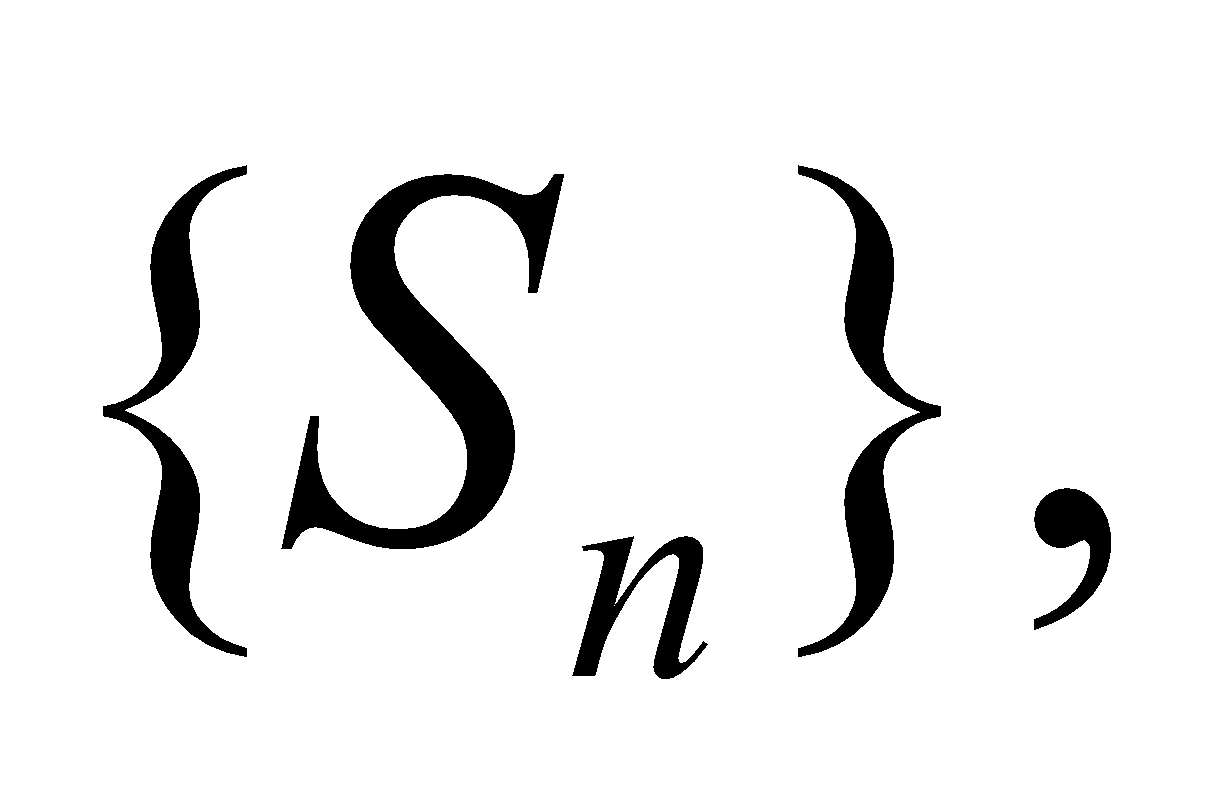where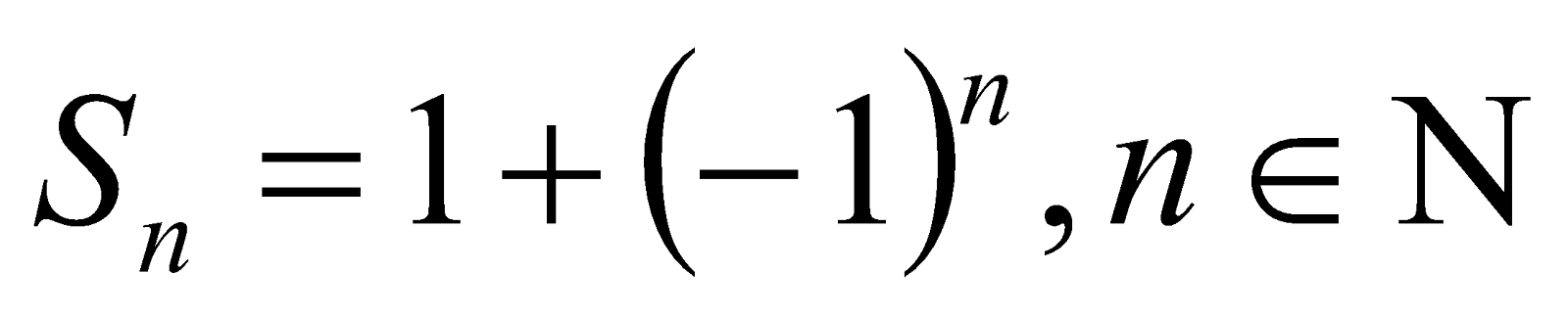(c) Write the bounds of the sequencewhere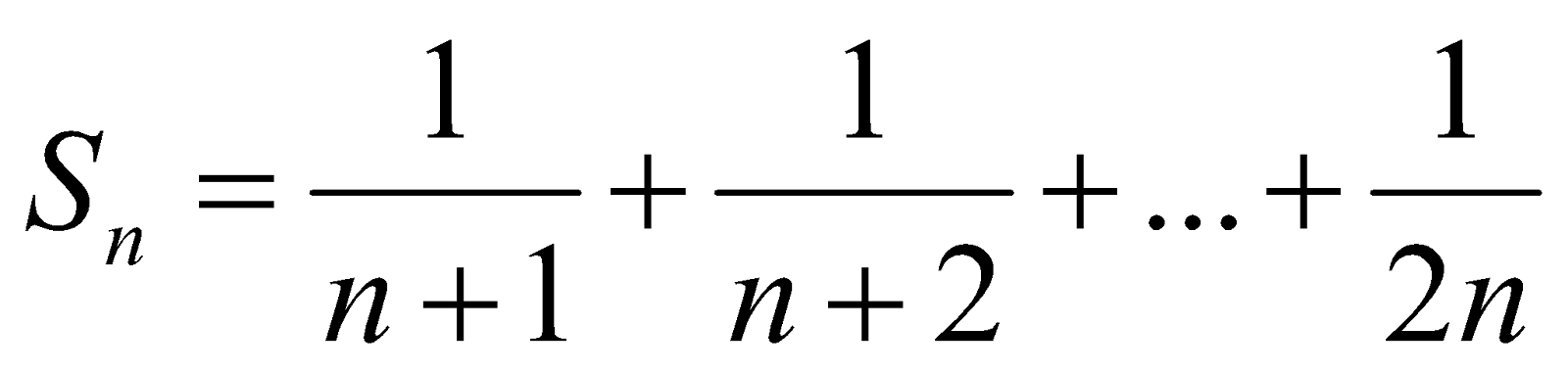Is a bounded sequence
(d) Show that a sequence converges to one limit point only.
Or
Show that every bounded sequence with a unique limit point is convergent.
2. (a) Write when an infinite series diverges.
(b) Write the statement of Leibnitz test for convergence of an alternating series.
(c) Test the convergence of the infinite series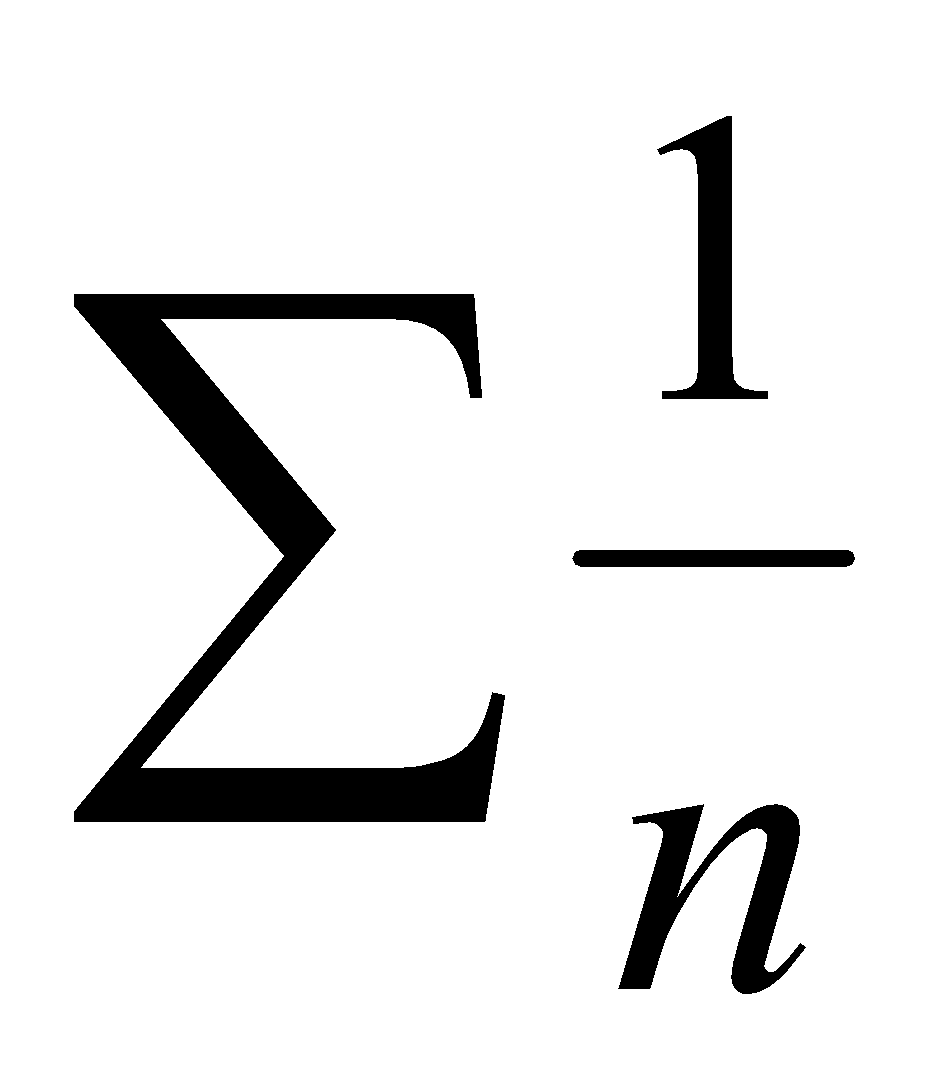.
(d) Test the convergence of the infinite series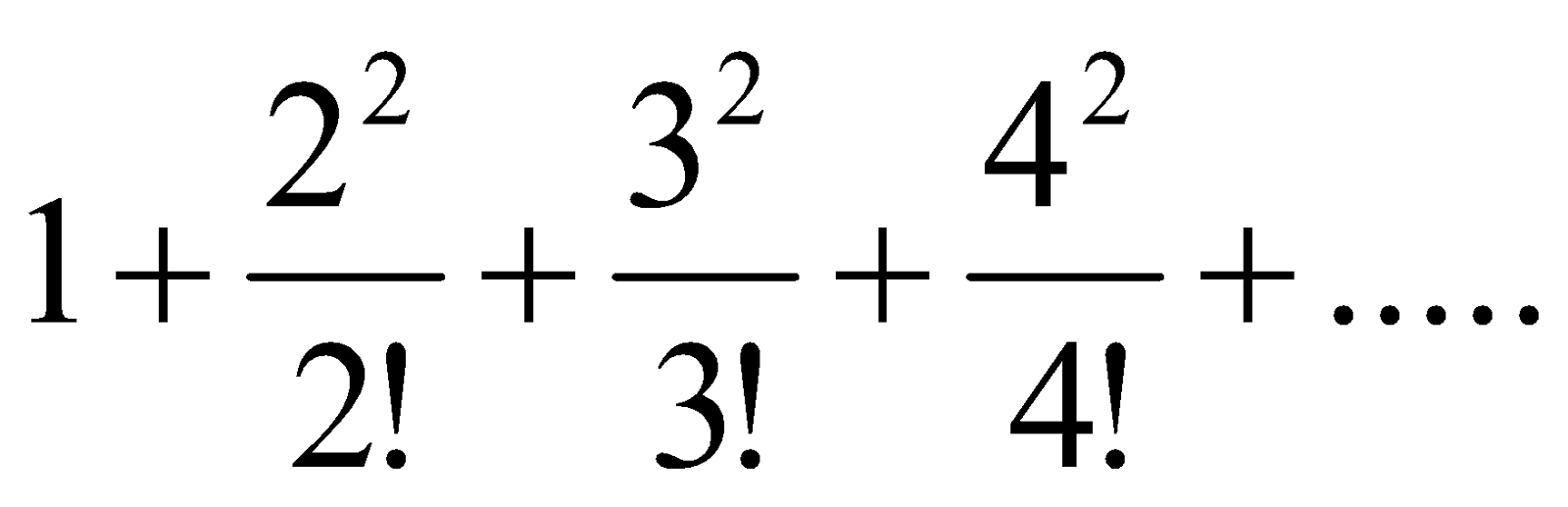(e) Show that if a series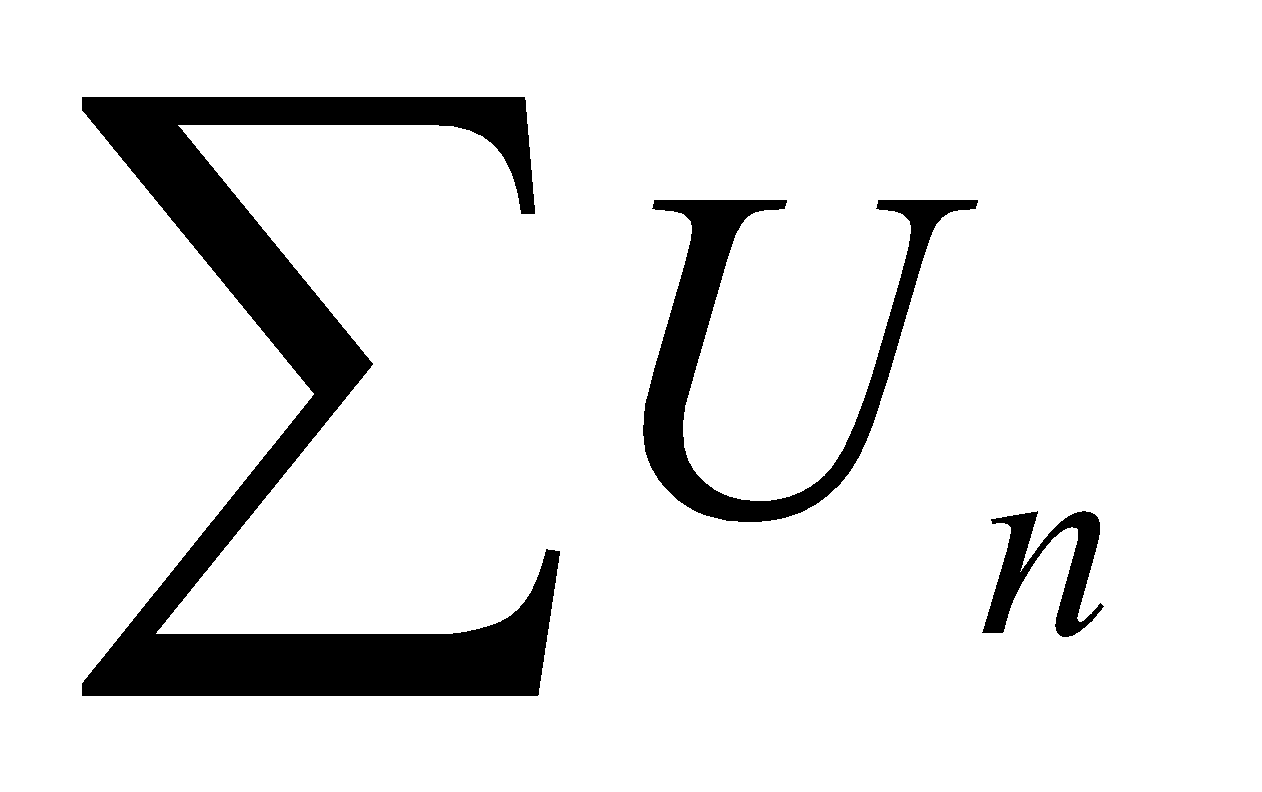of positive monotonic decreasing terms converges, then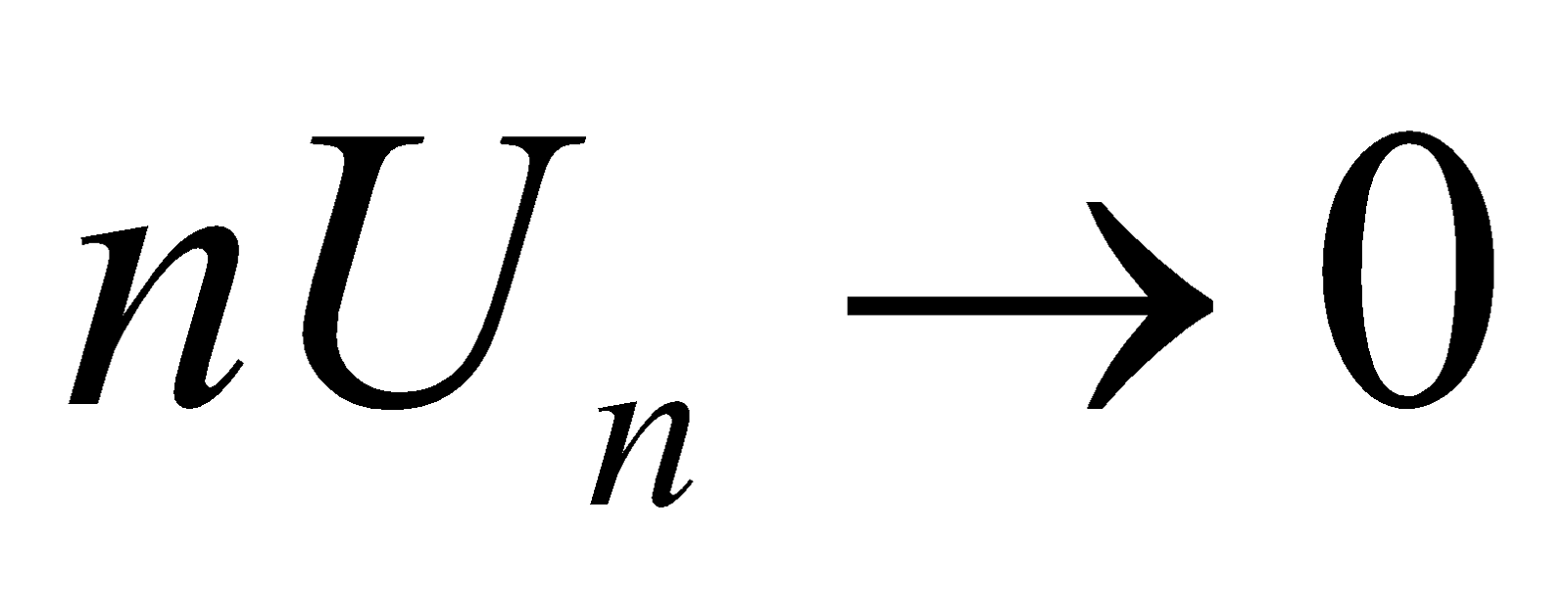as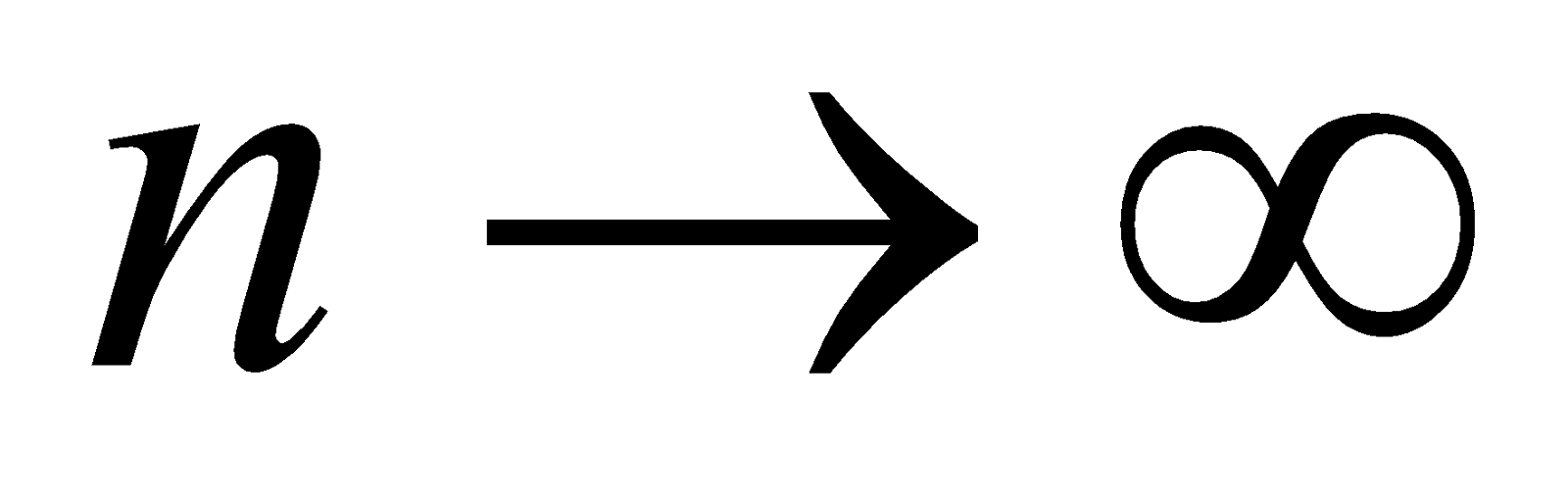.
Or
Test the convergence of the infinite series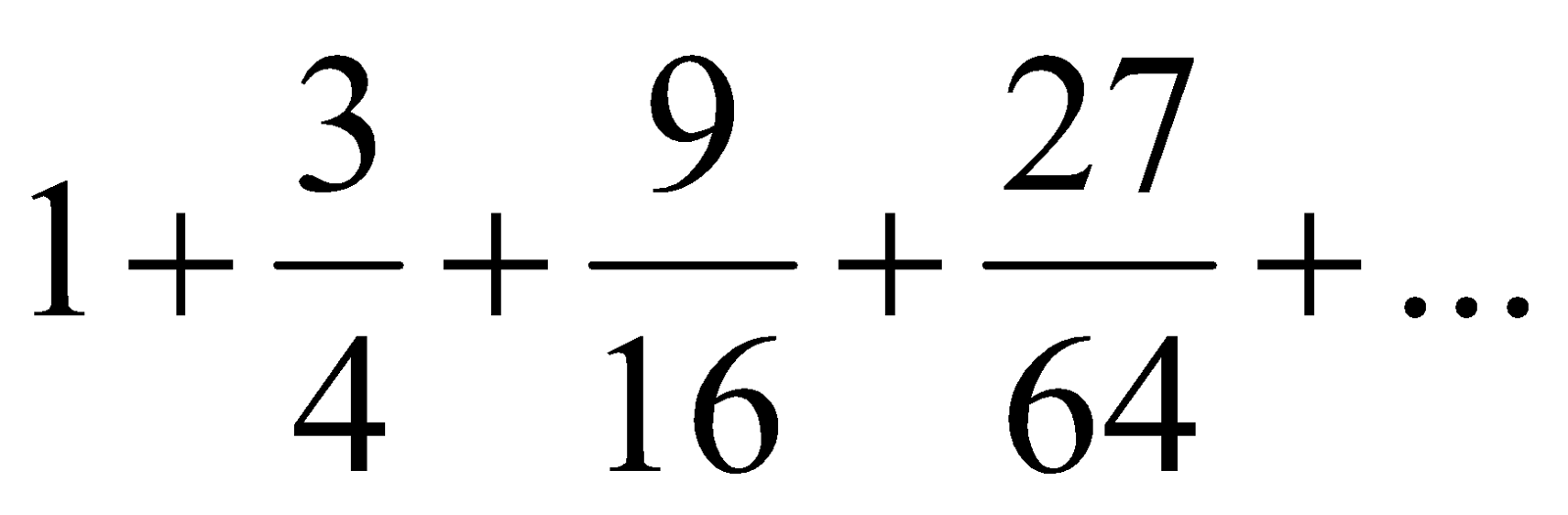.
3. (a) Ifbe a root of an equation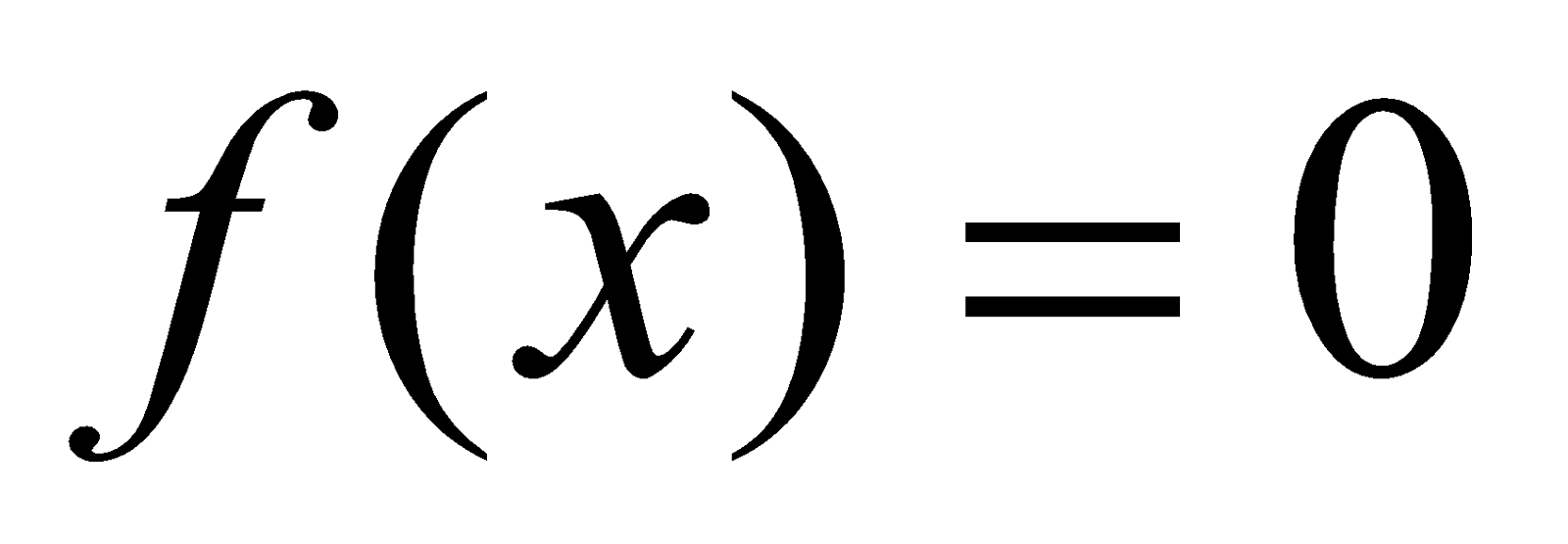, then write a factor of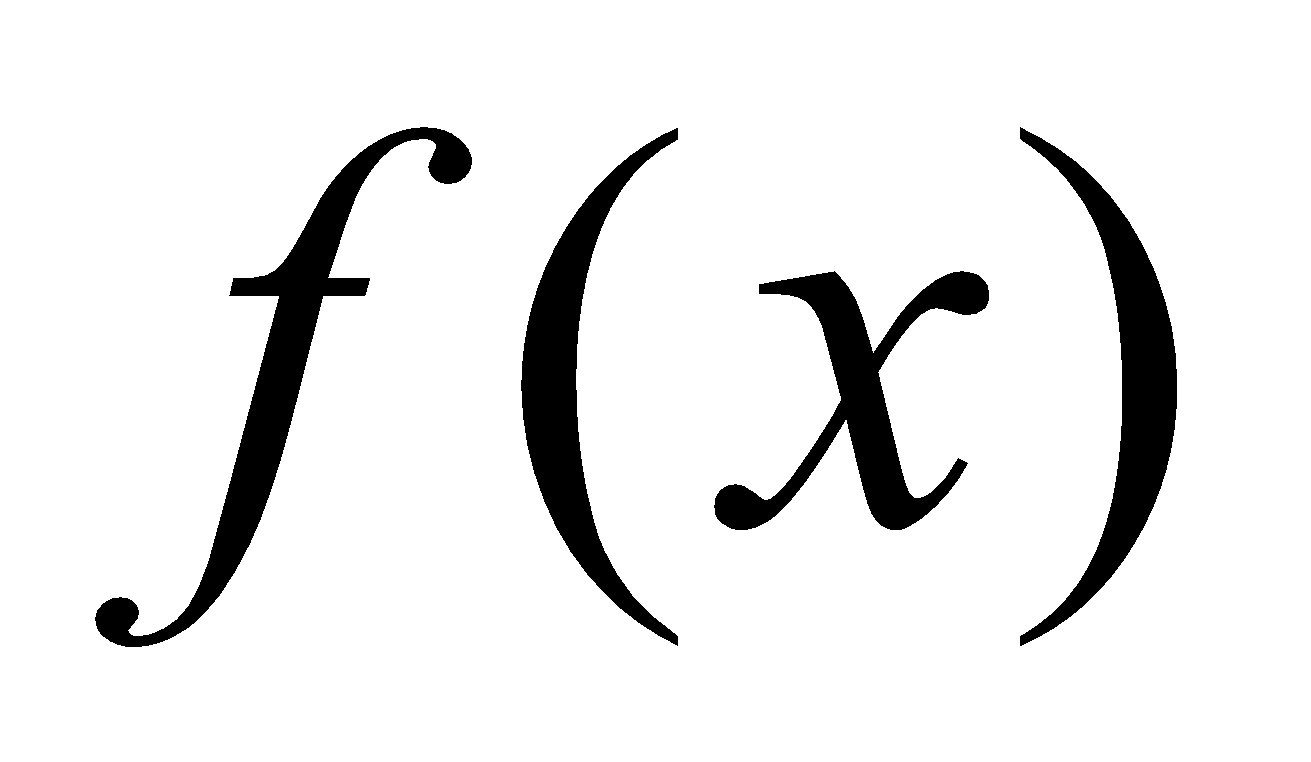. 1
(b) If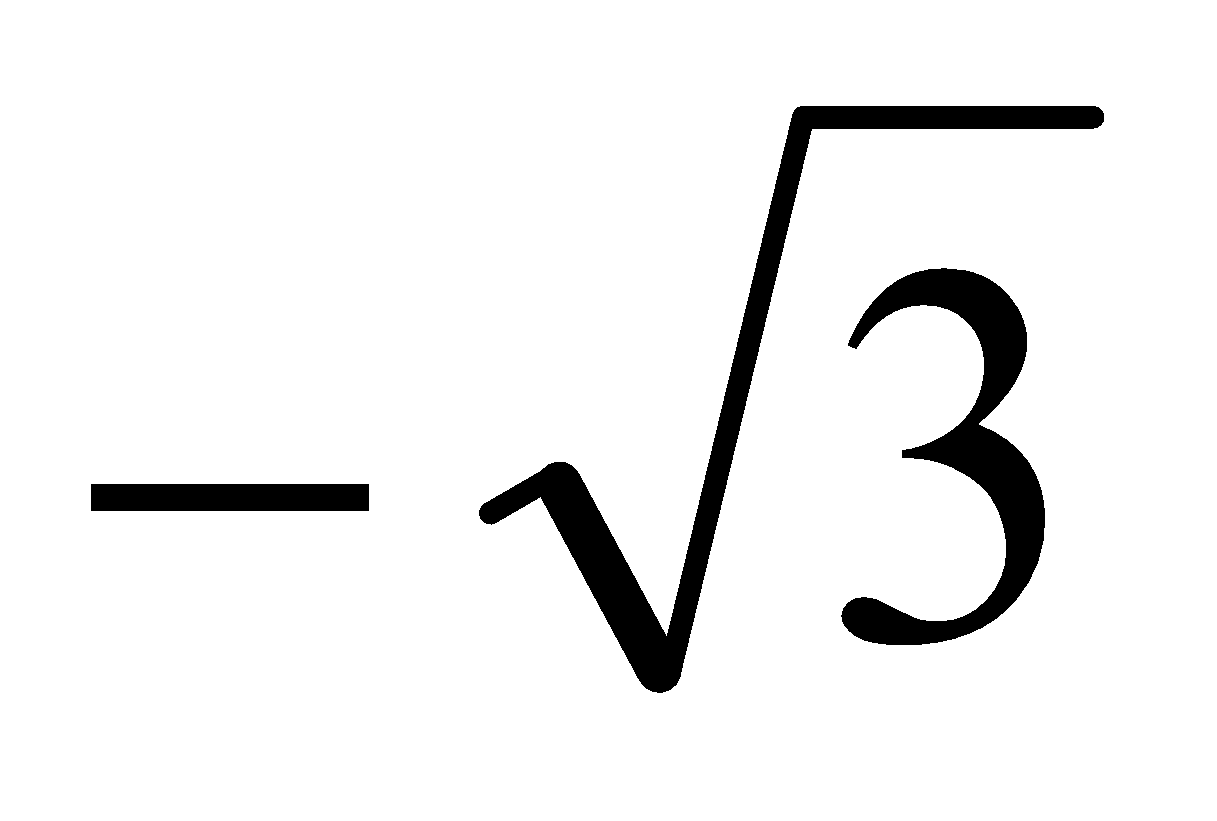and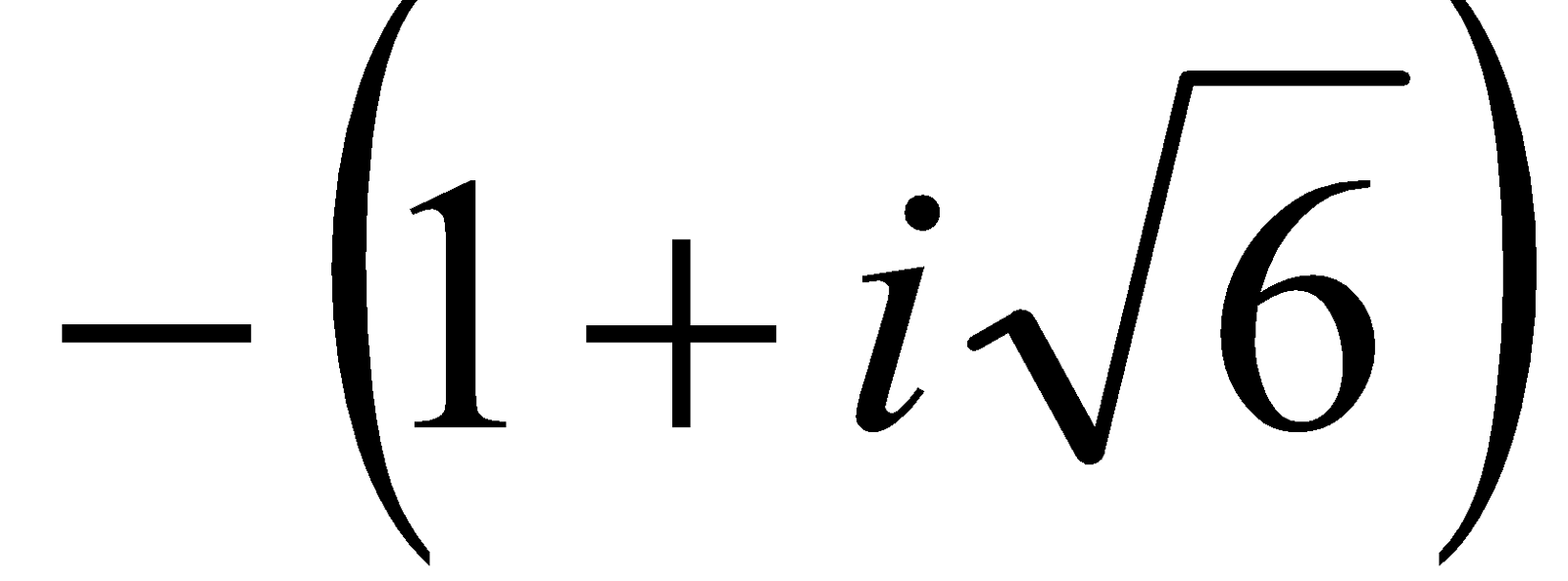are two roots of the equation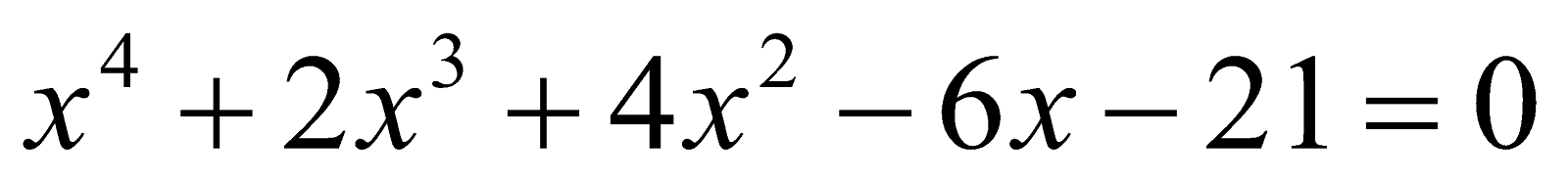, then write the other two roots of the equation. 1
(c) Write the nature of the roots of the equation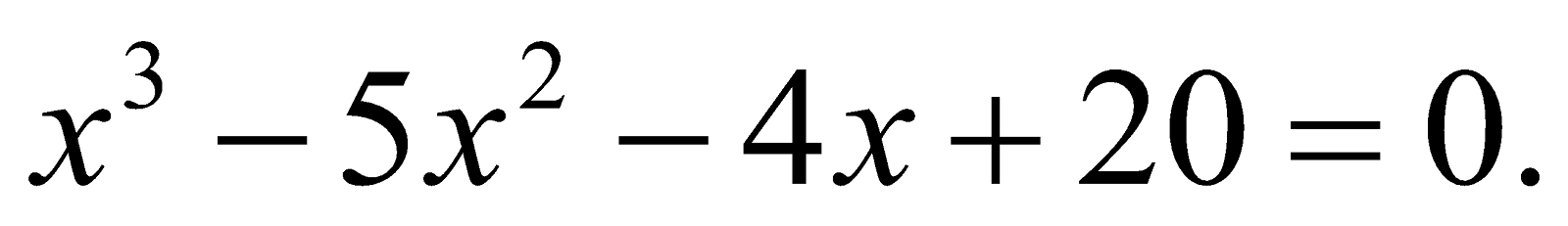2
(d) If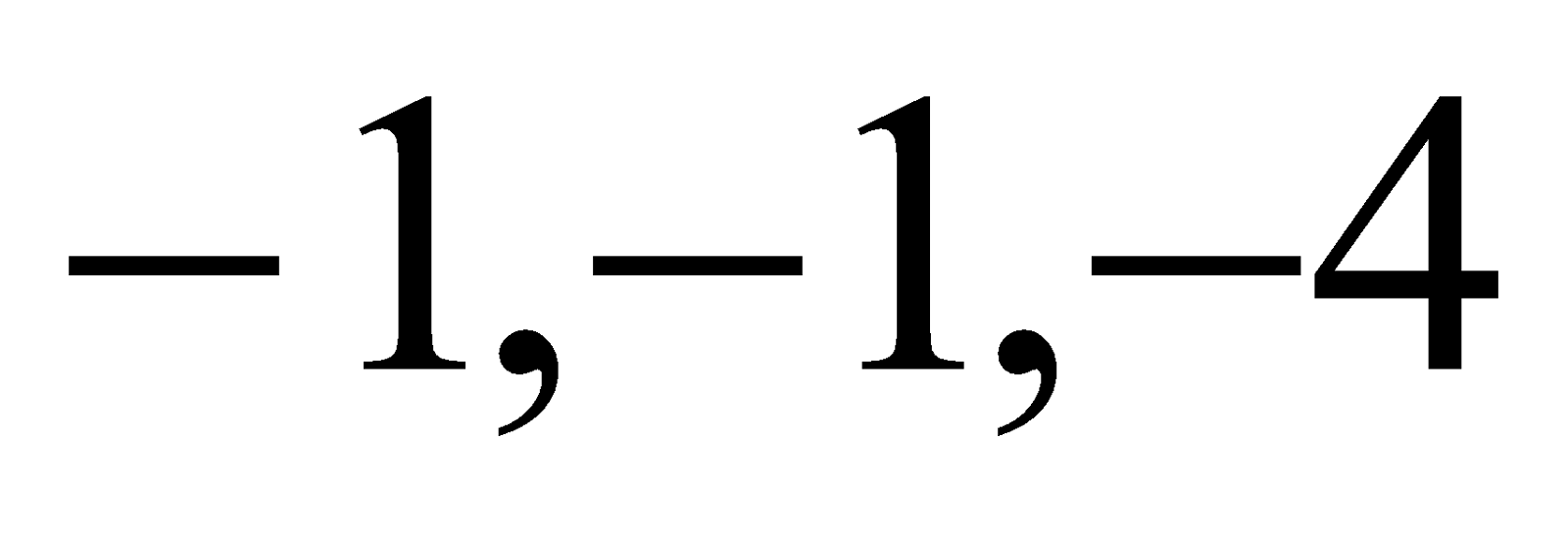are the roots of the equation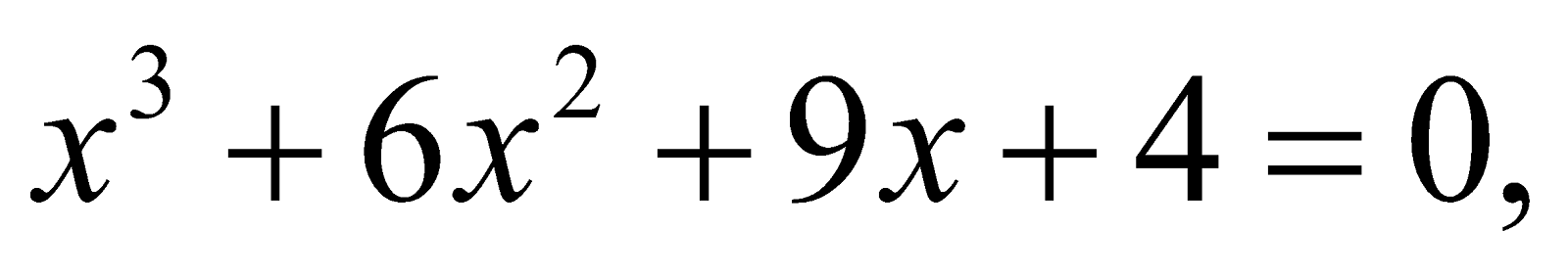then write the equation whose roots are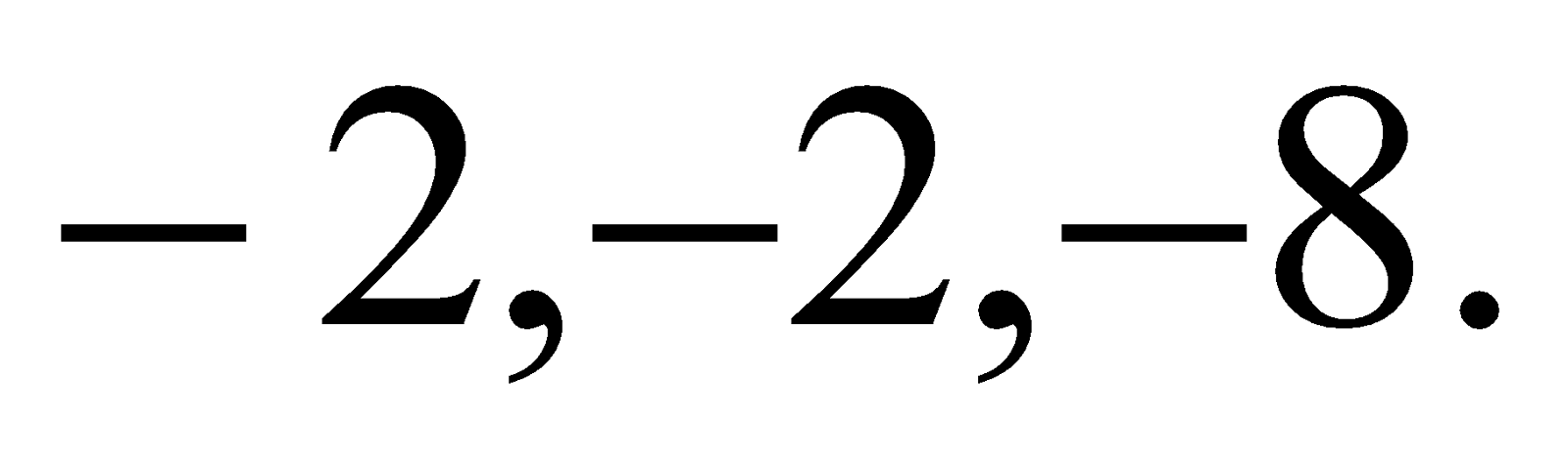2
(e) If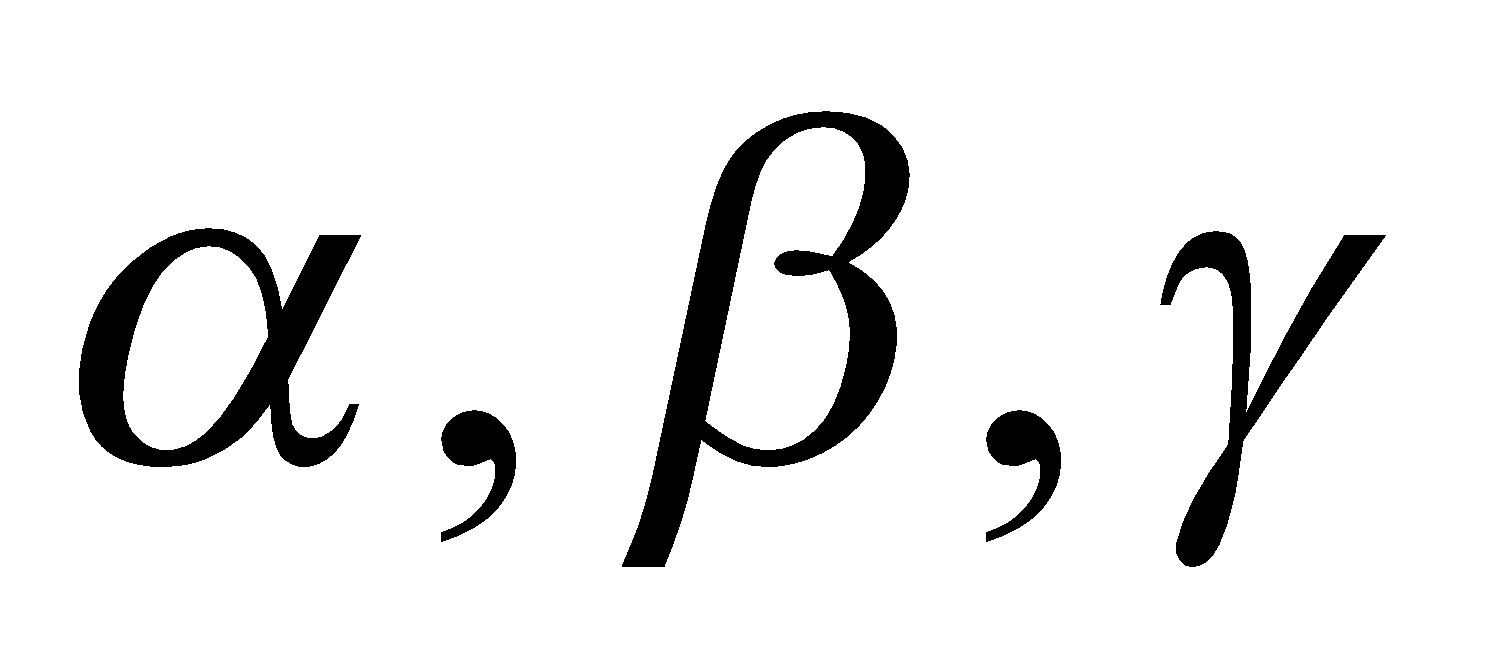be the roots of the equation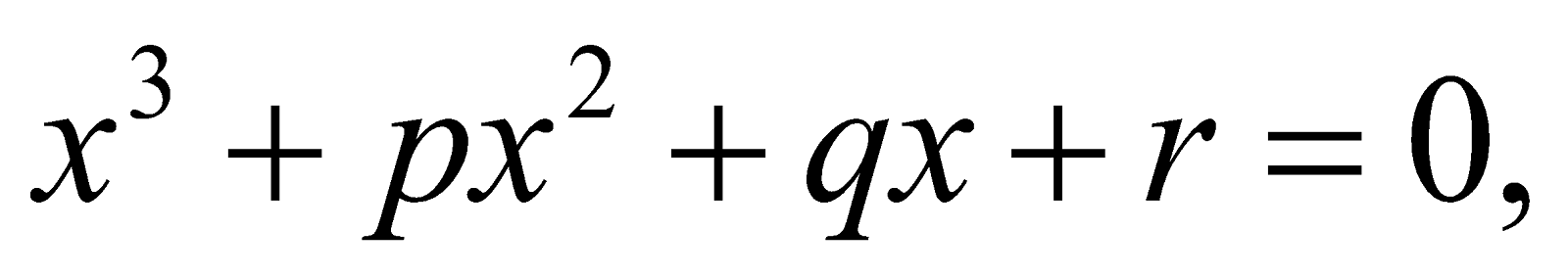then find the value of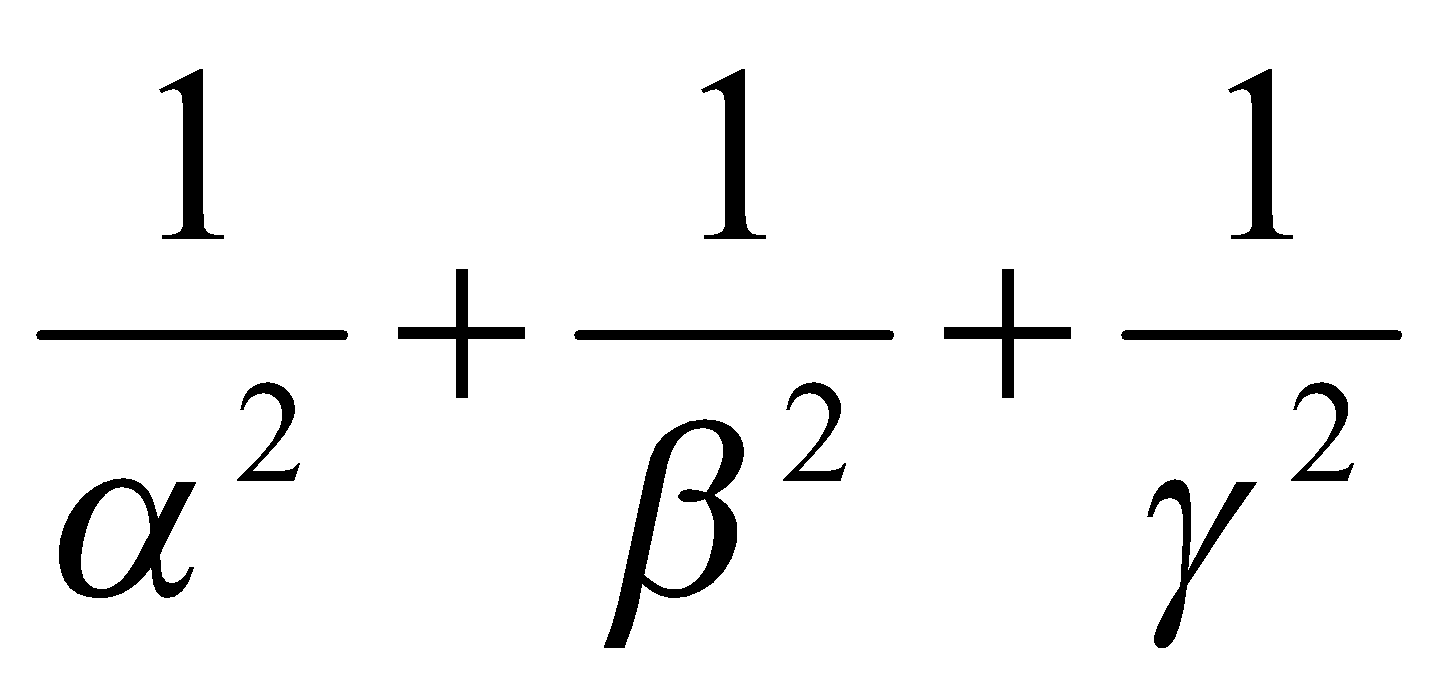3
(f) Solve the equation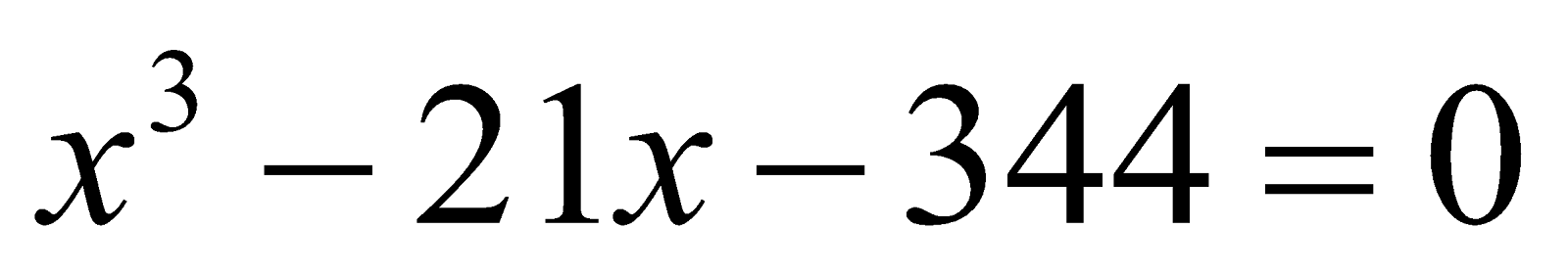by Cardan’s method. 6
Or
Ifbe the roots of the equationthen find the value of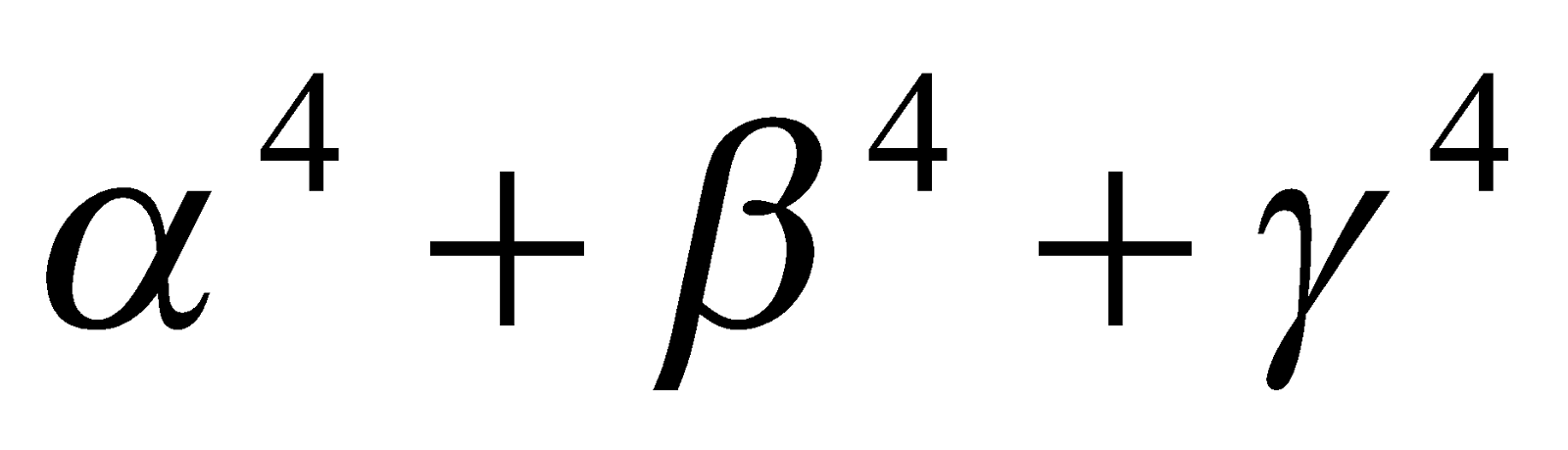.

GROUP – B
(Trigonometry)

4. (a) Choose the correct answer: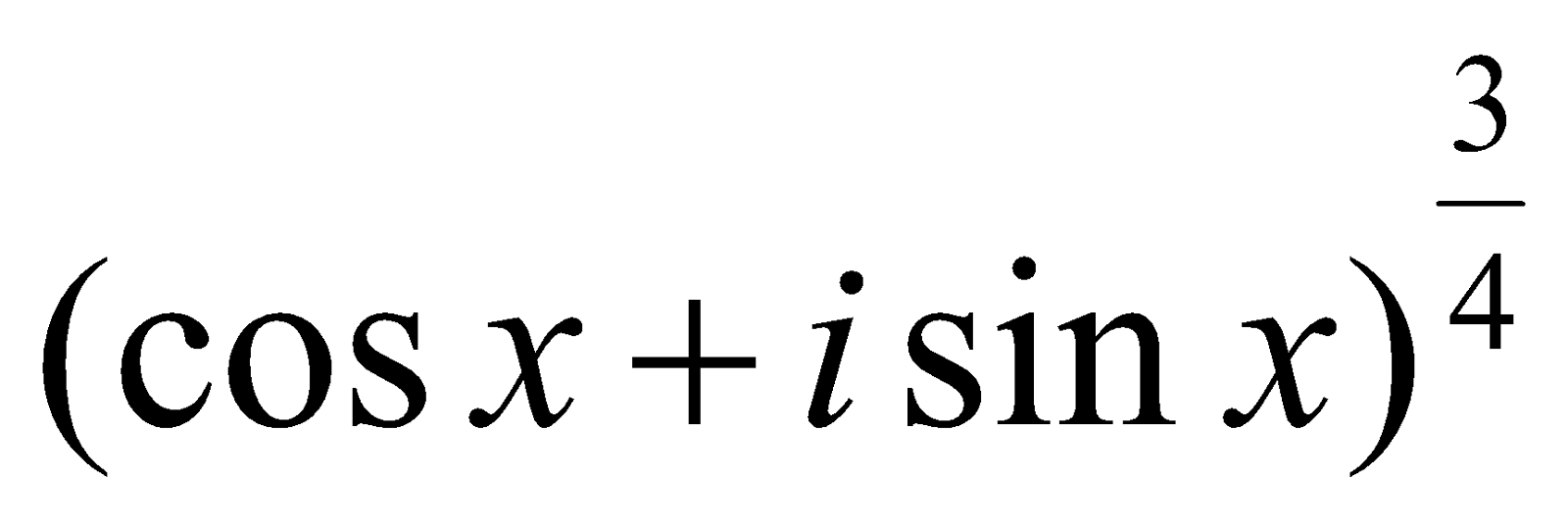is equal to
1.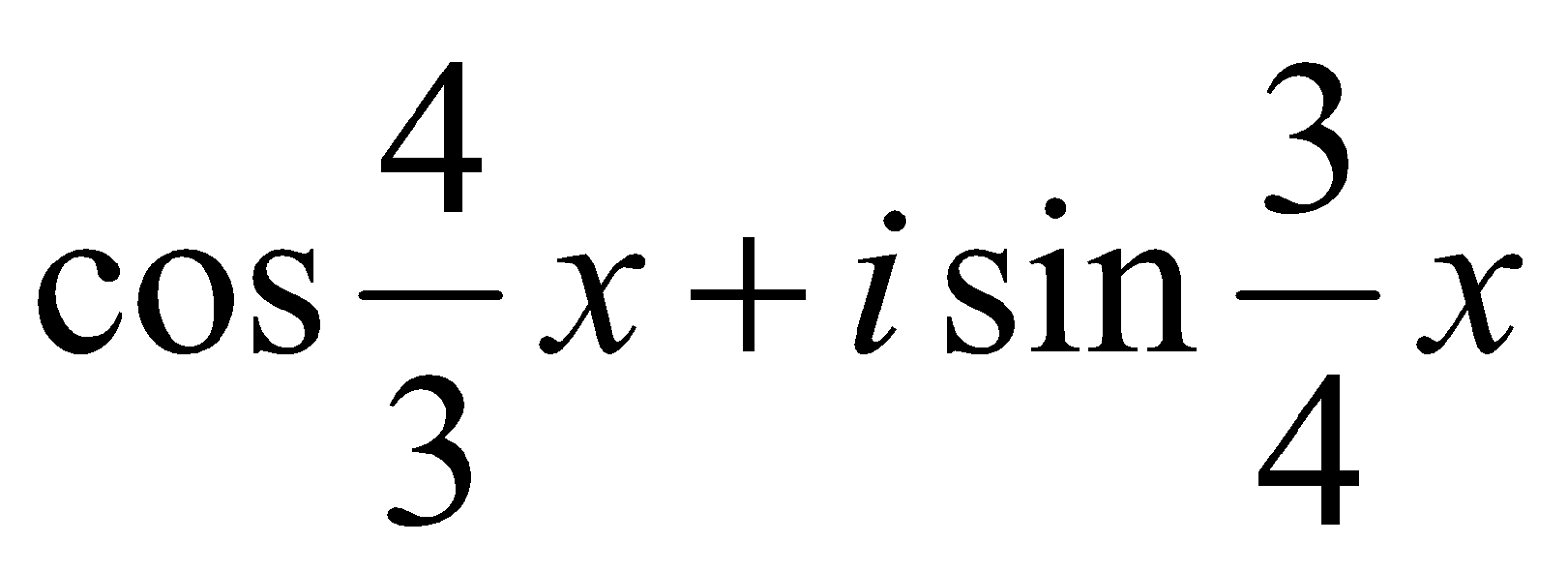2.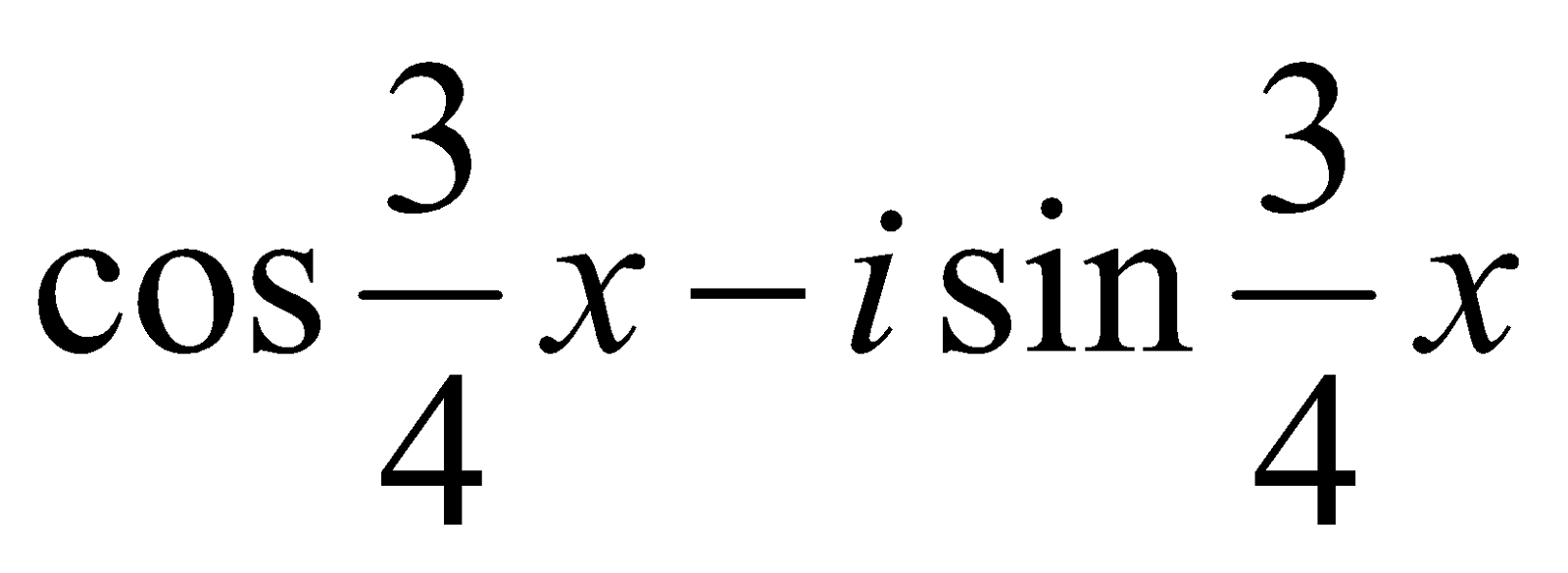3.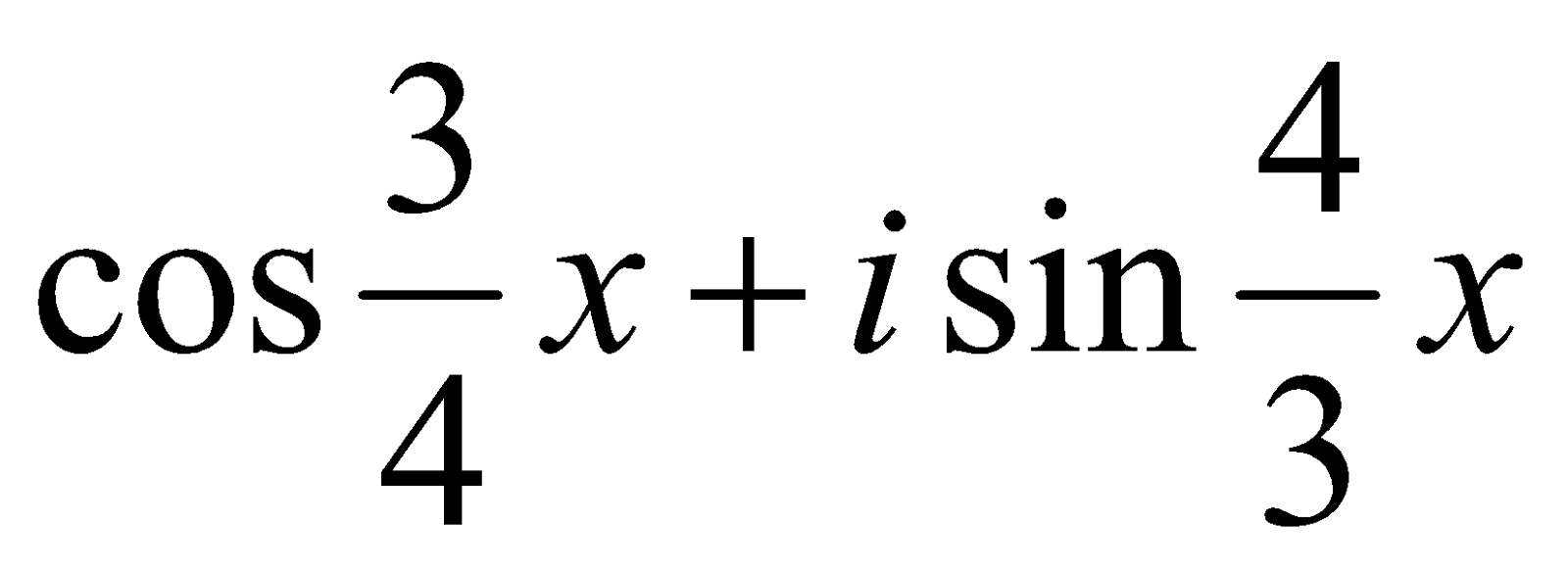4.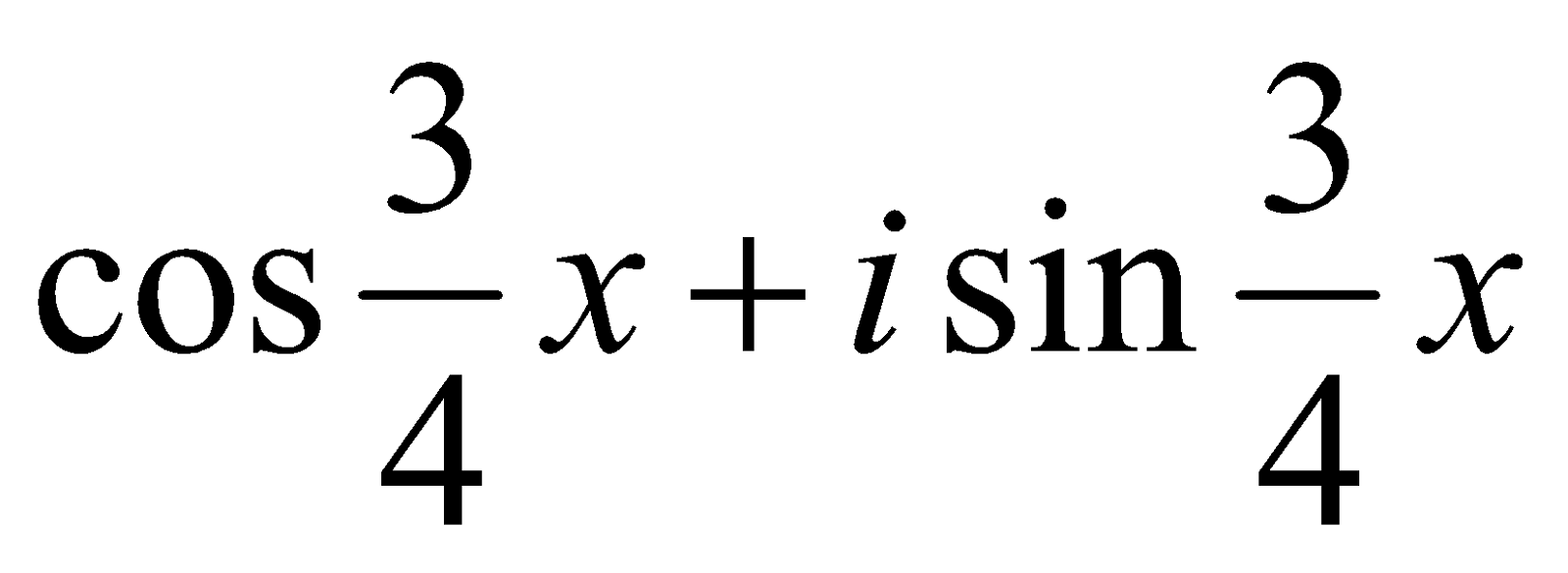(b) Write the roots of the quadratic equation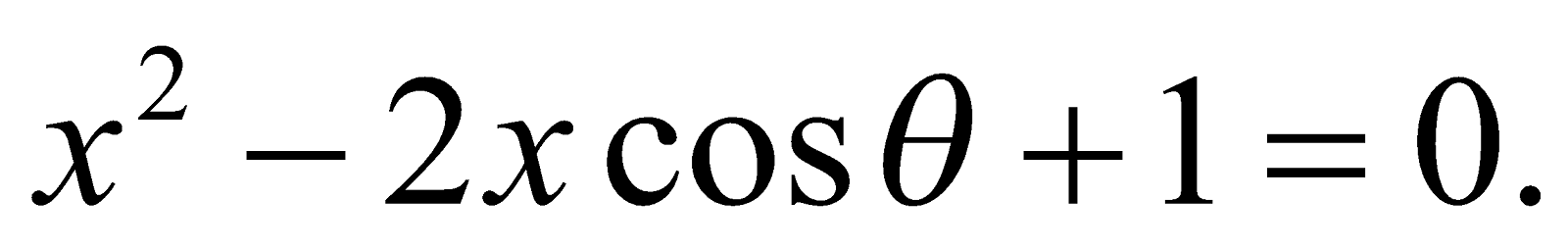(c) Express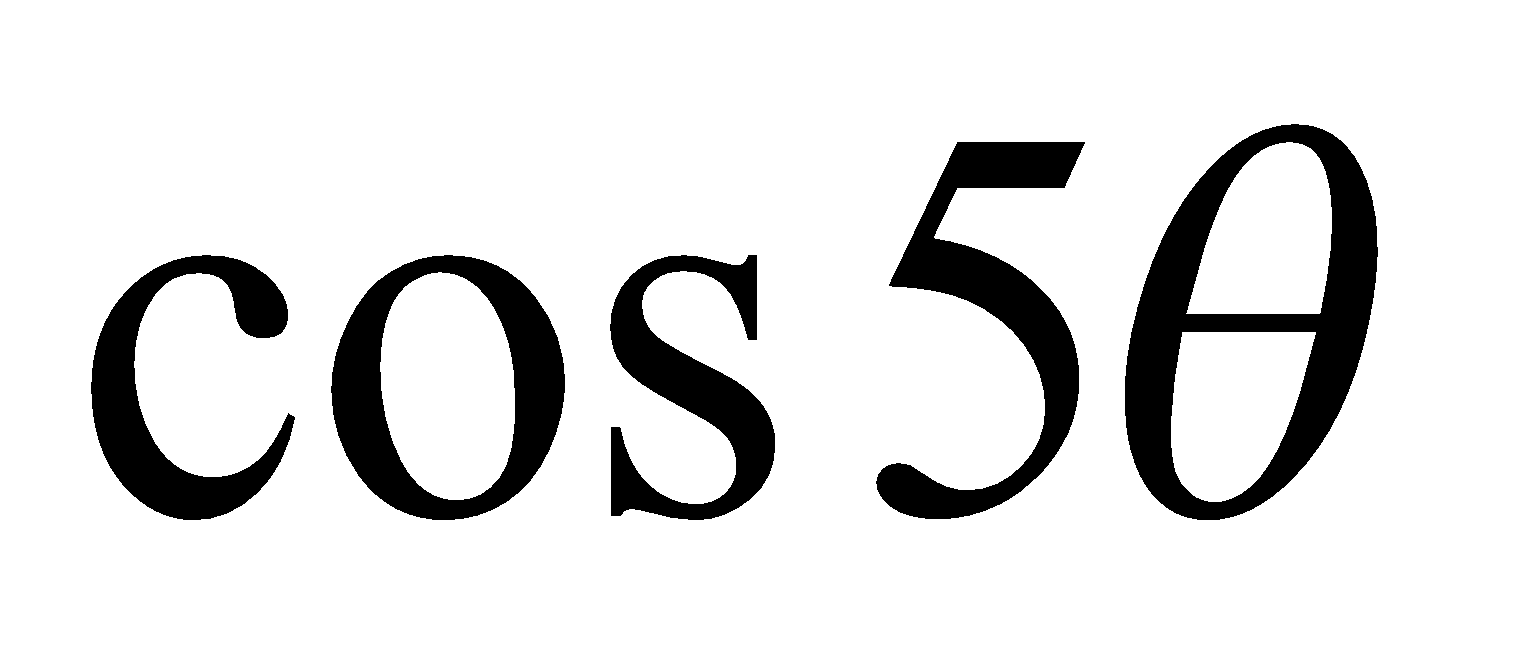in powers of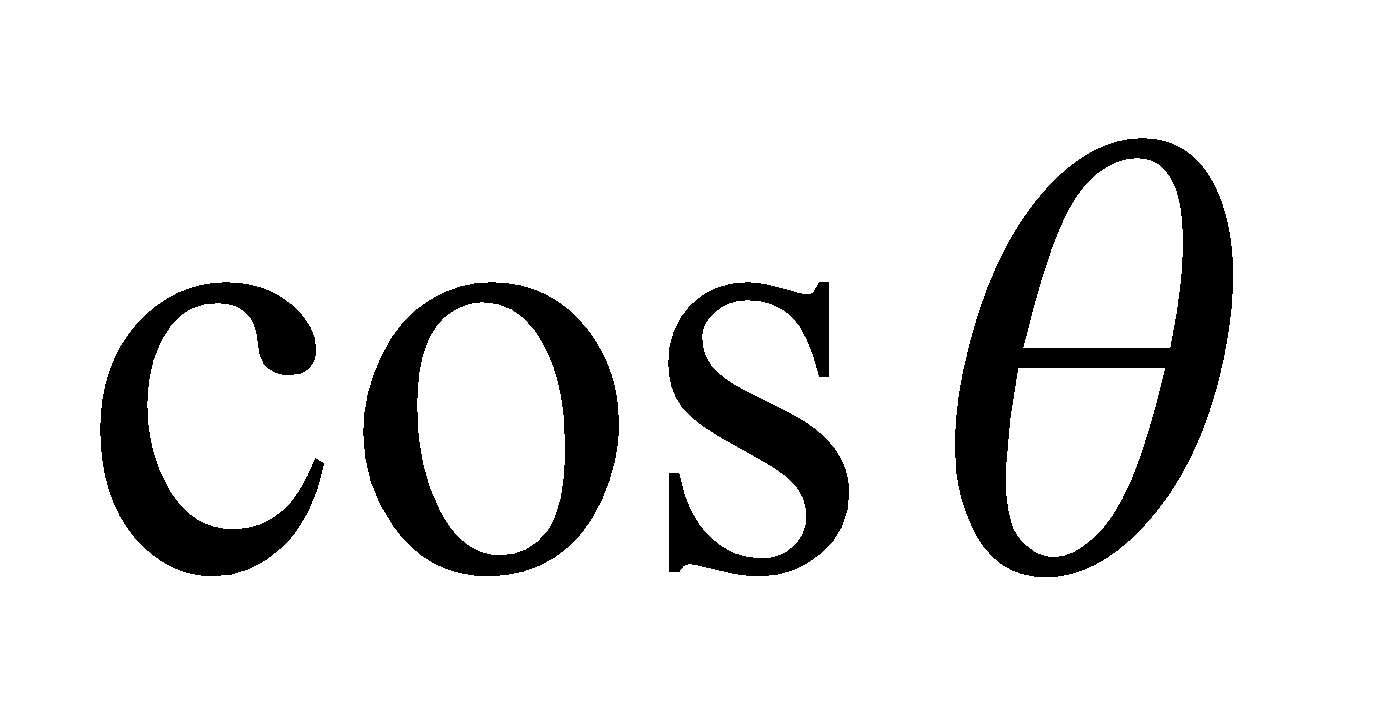.
Or
If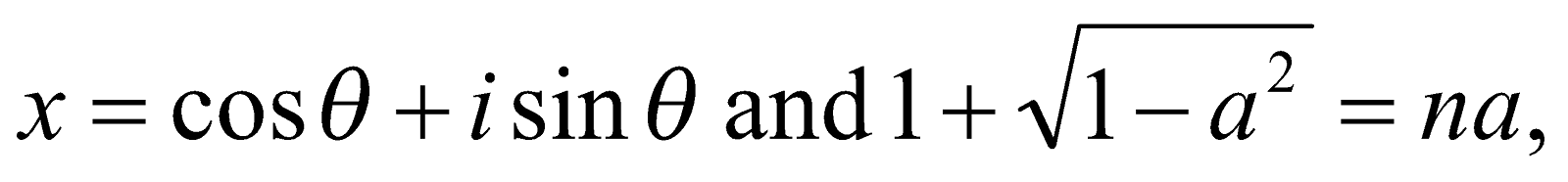prove that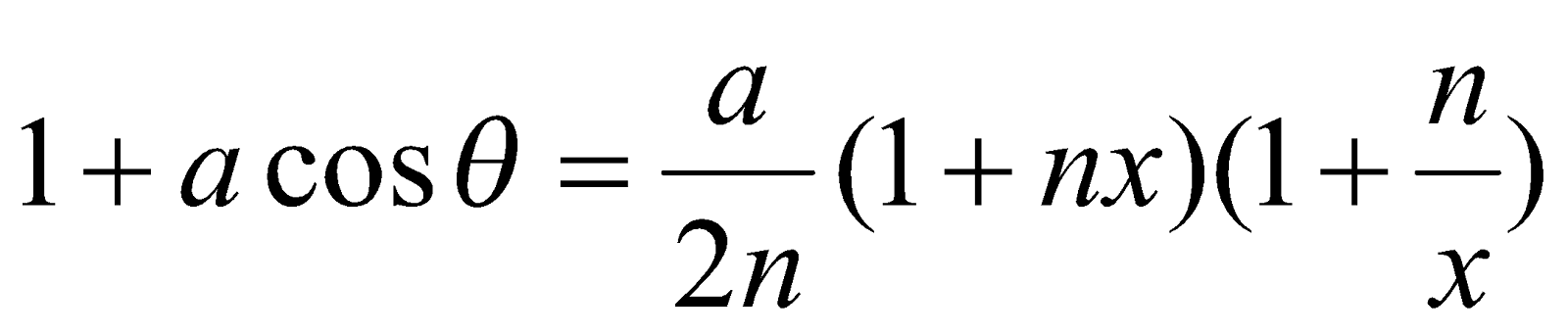5. (a) Show that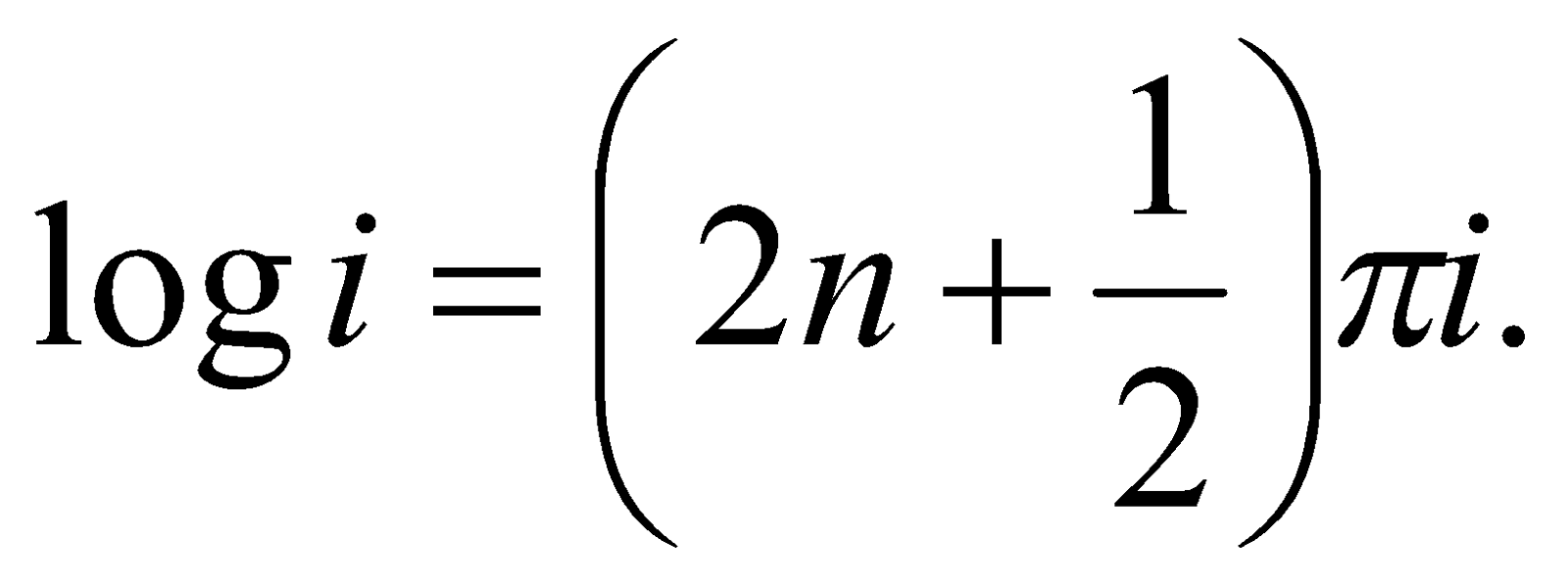2
(b) Show that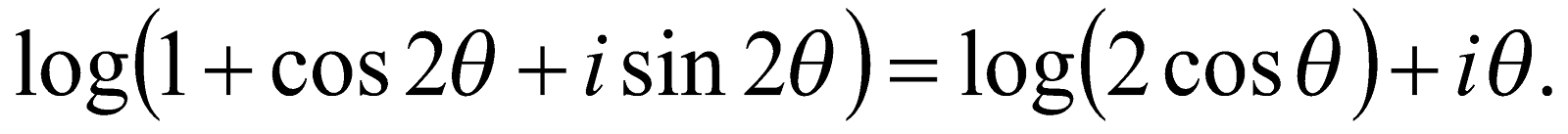3
Or
Expressin the form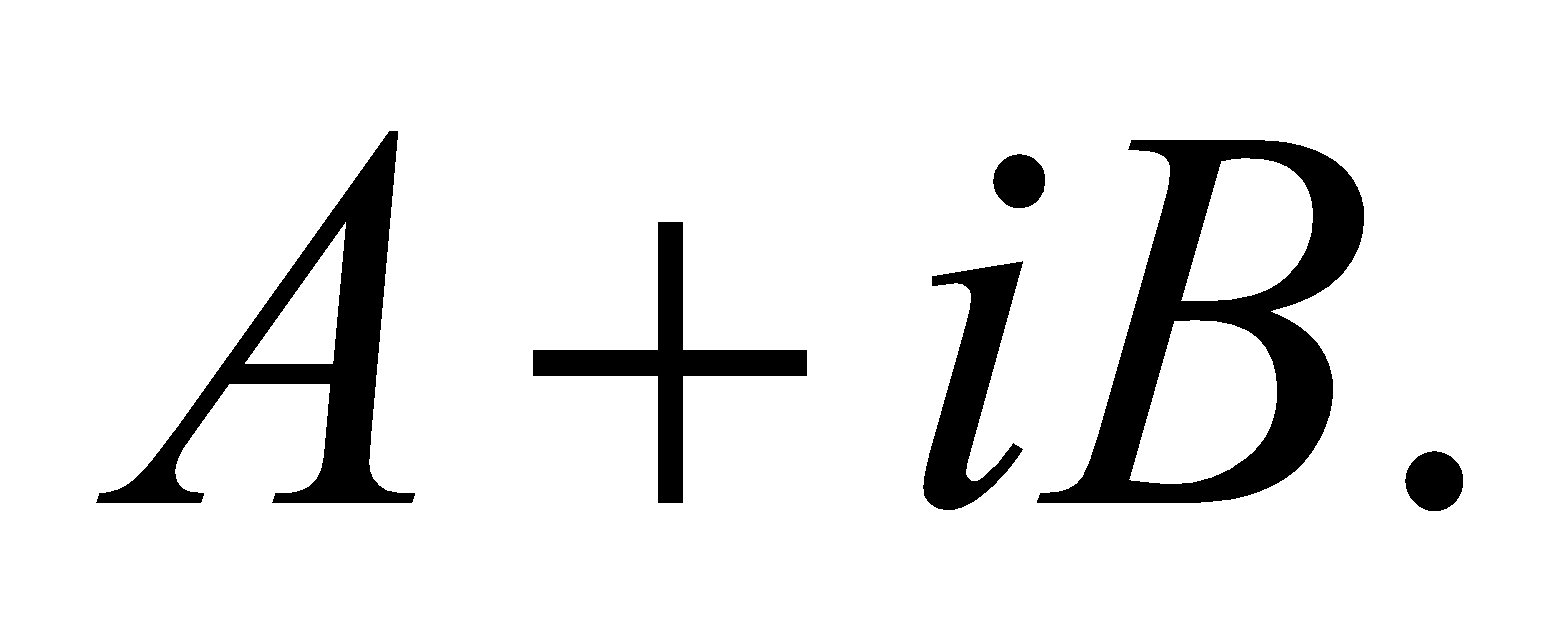6. (a) Write the interval of x for which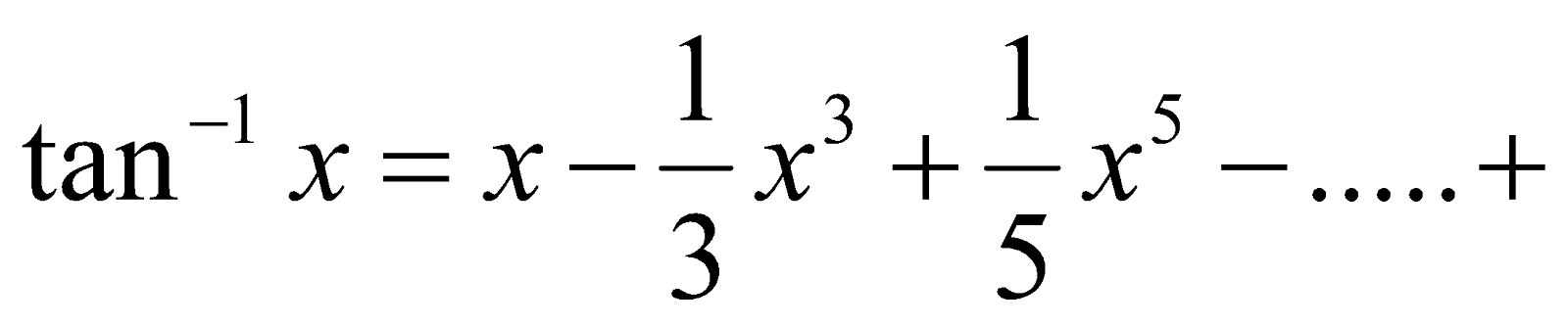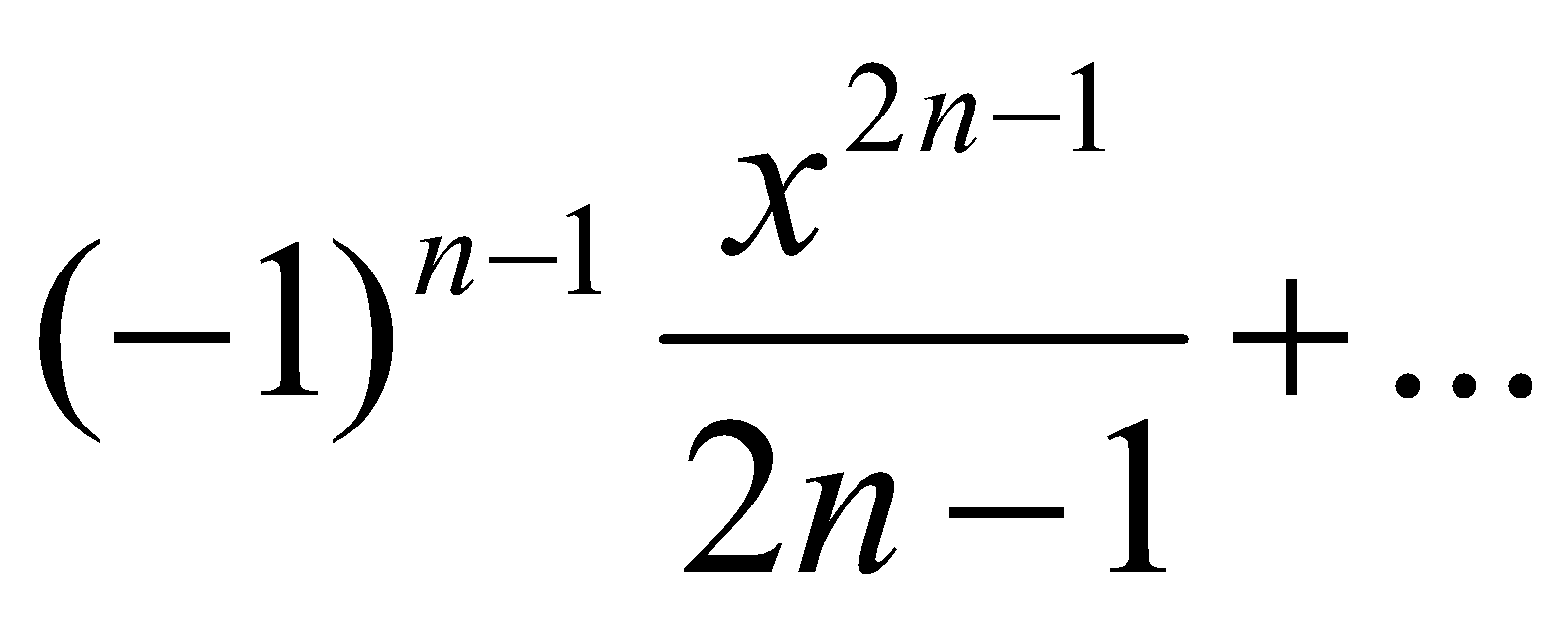1
(b) Show that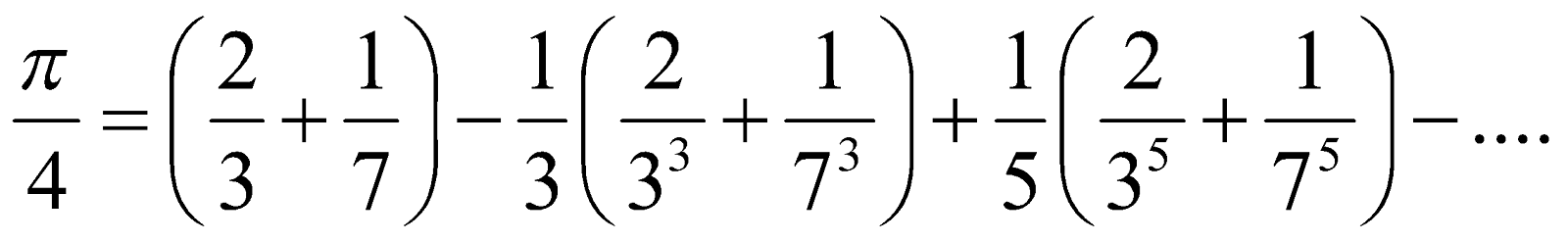3
Or
Expand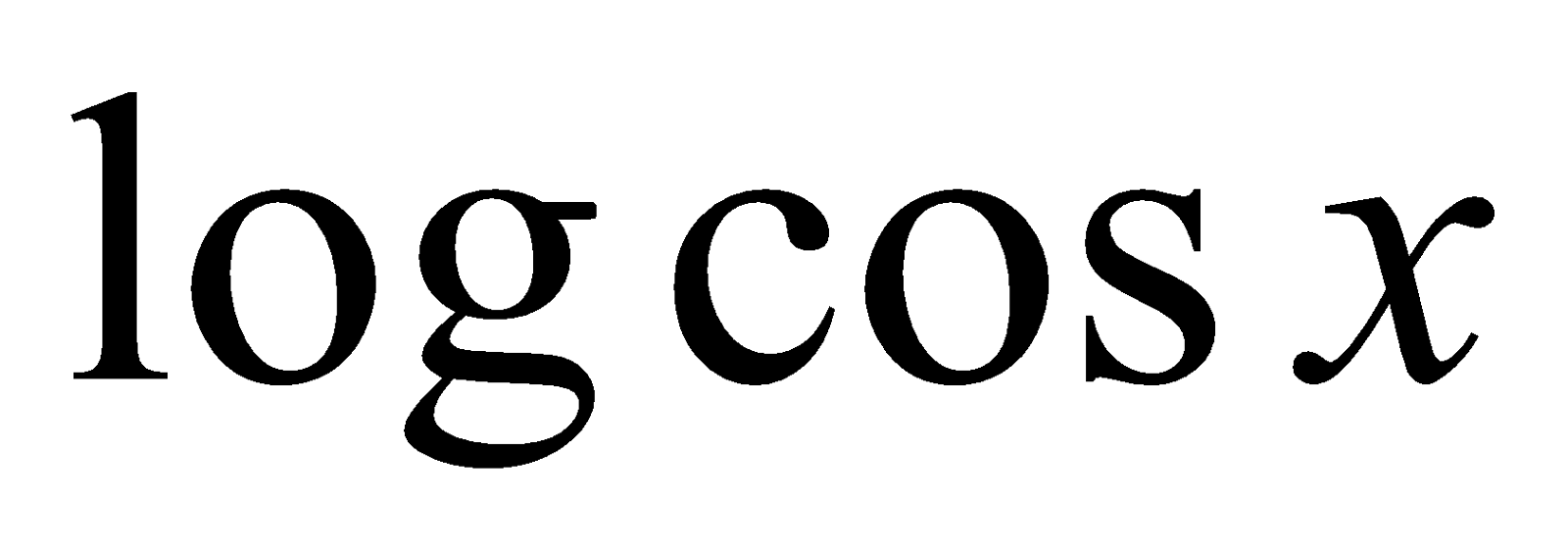in ascending powers of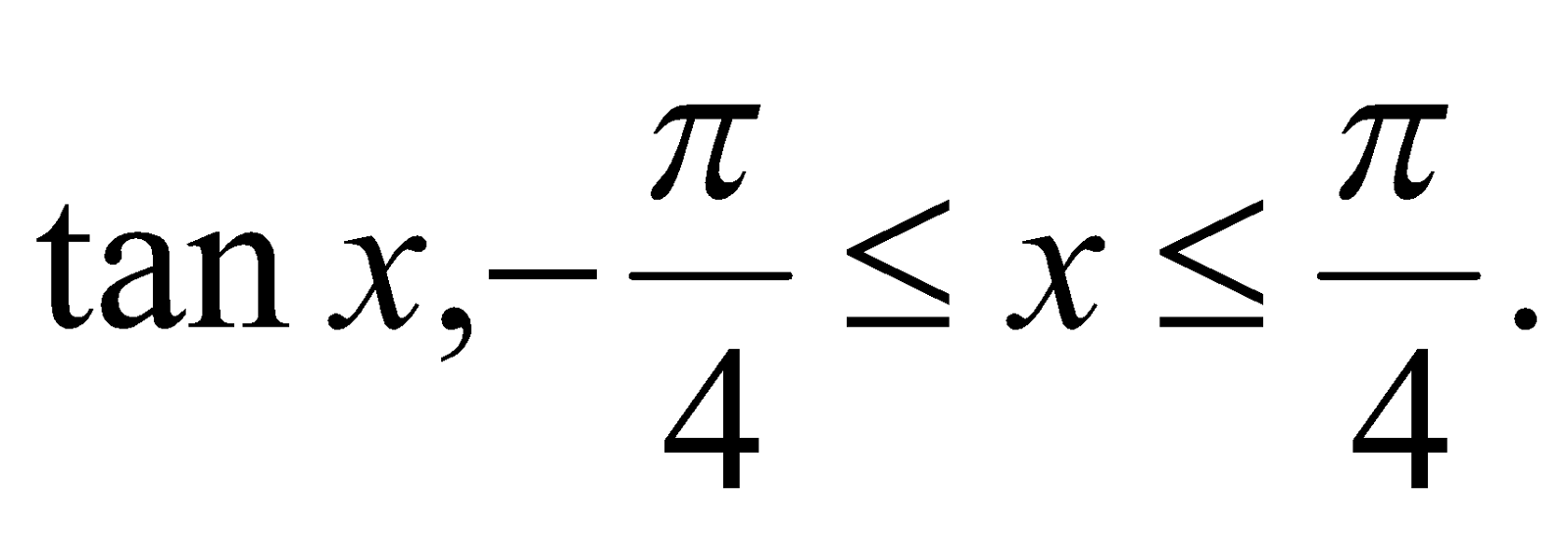7. (a) Choose the correct answer: 1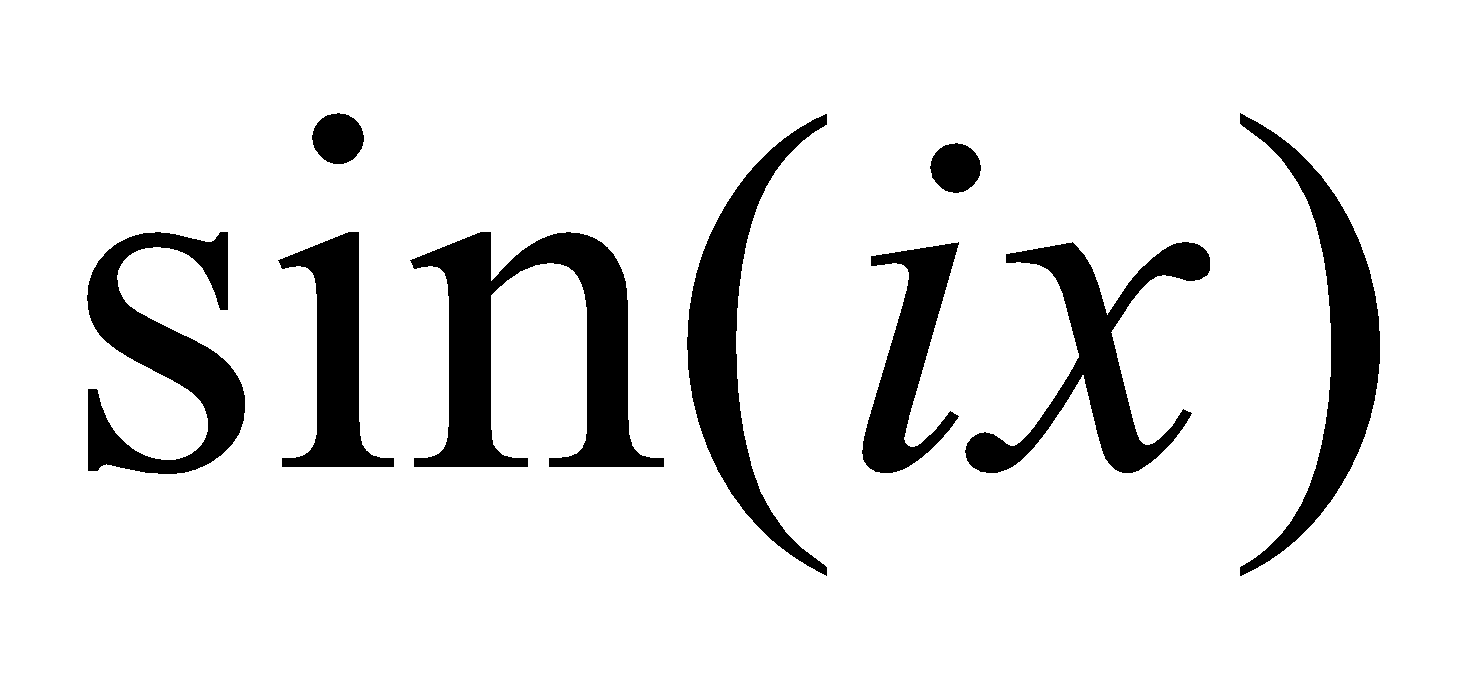is equal to
1.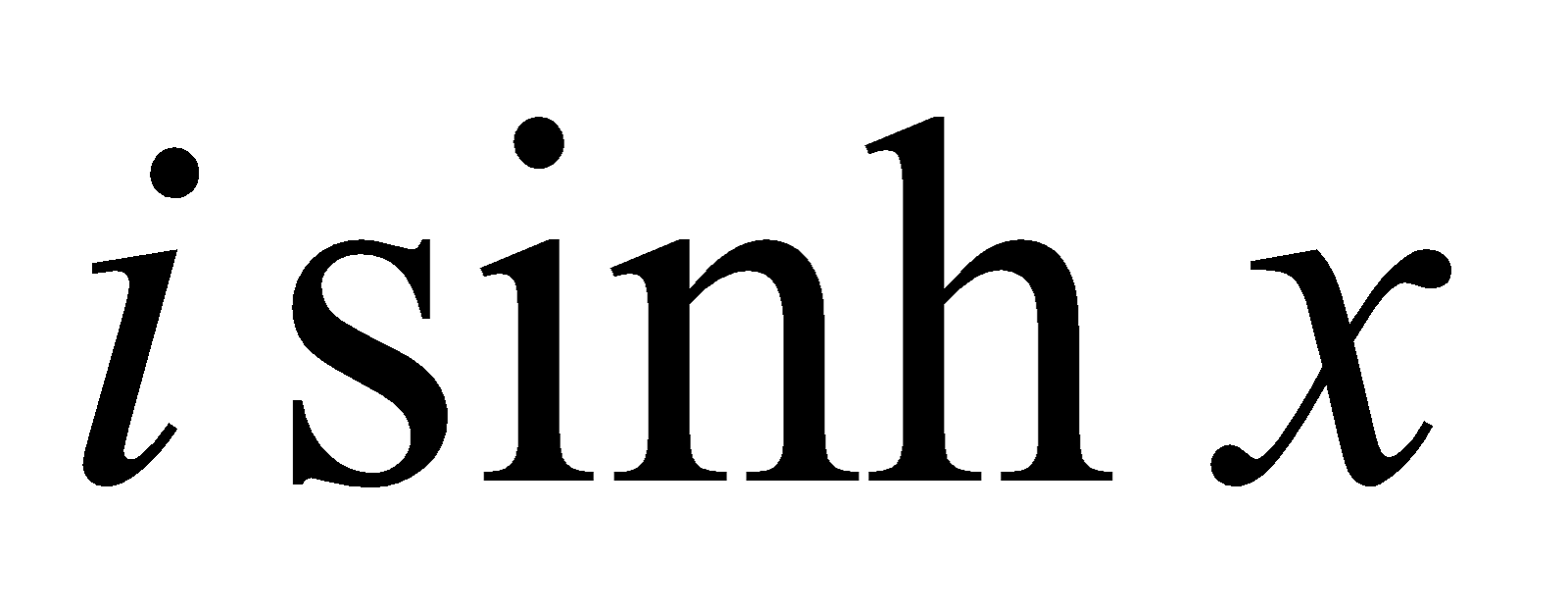2.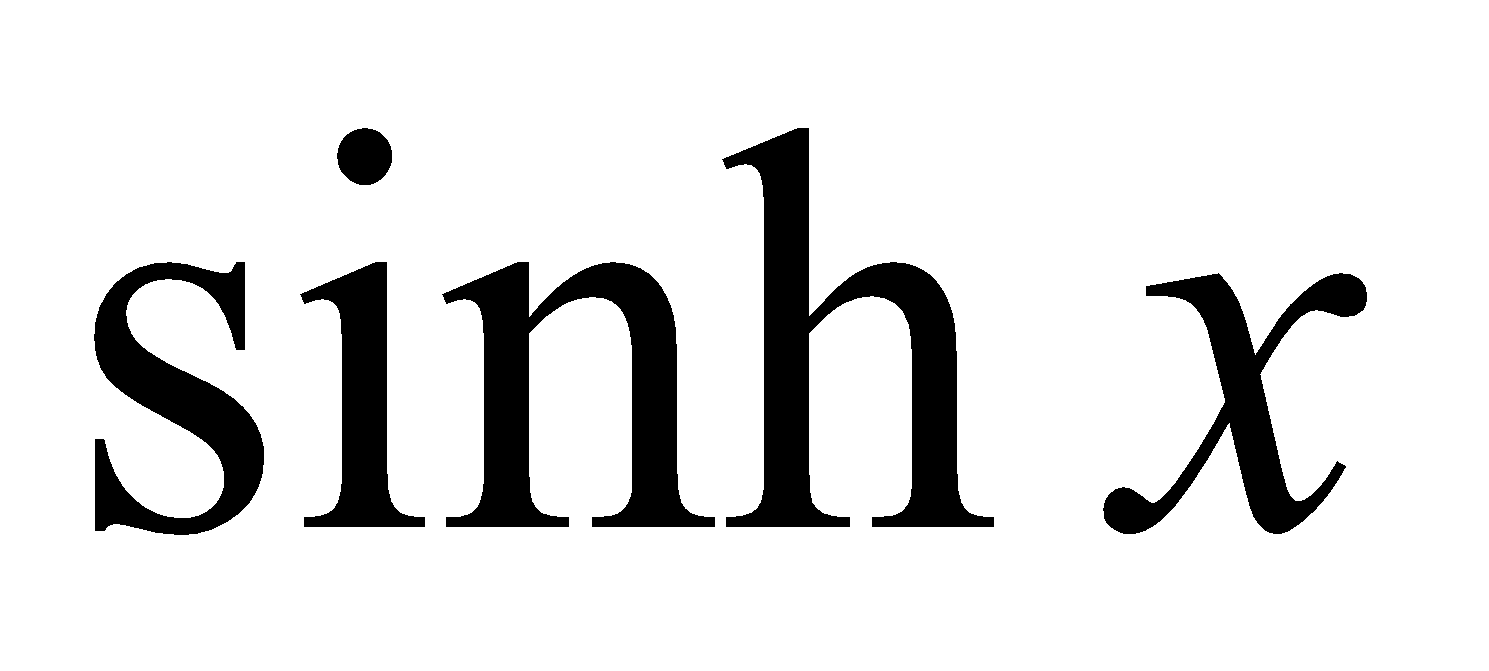3.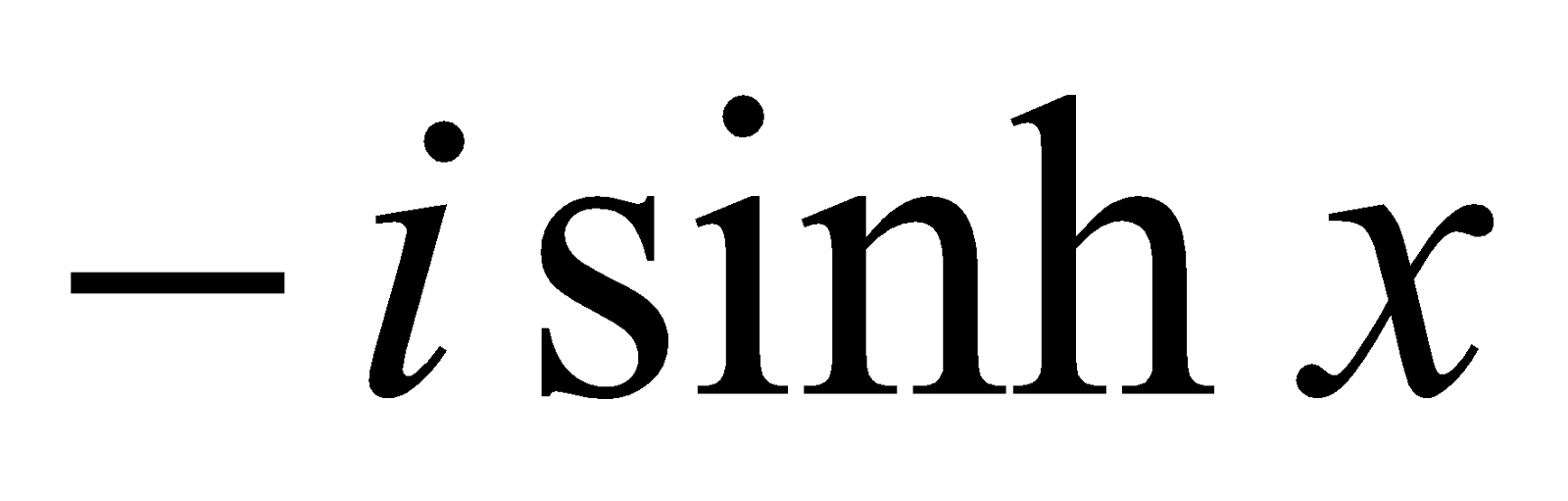4.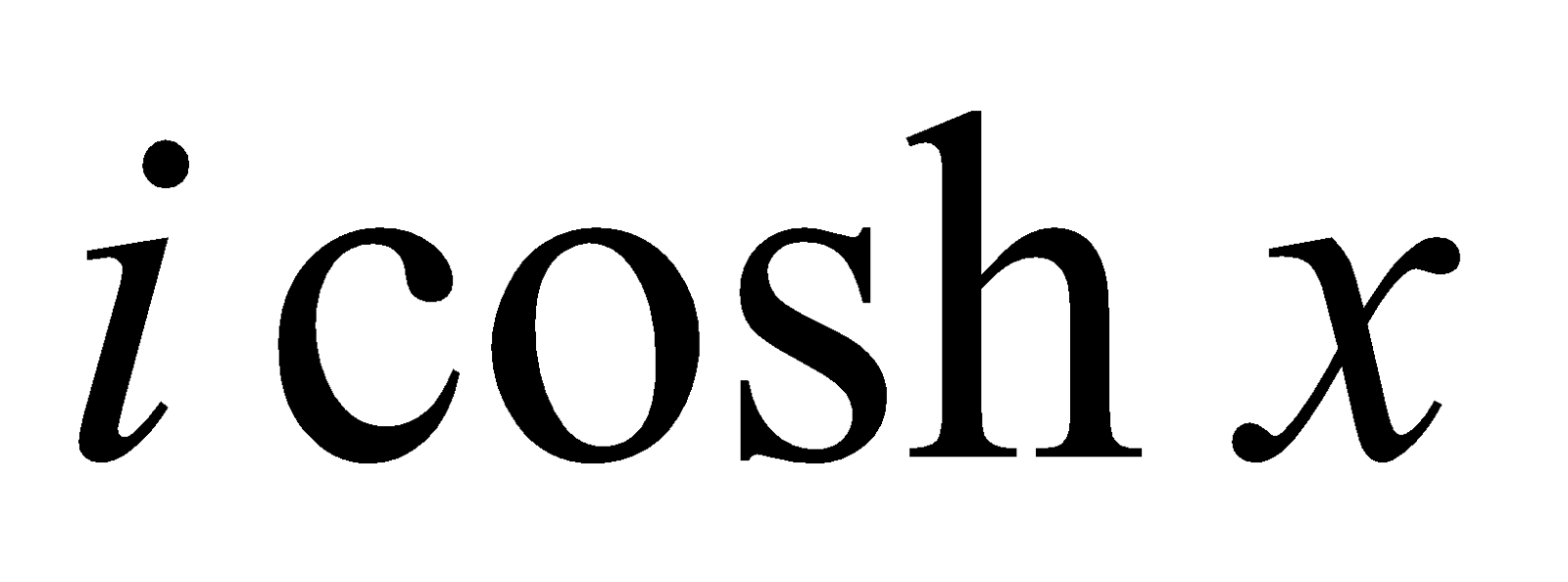(b) Write the period of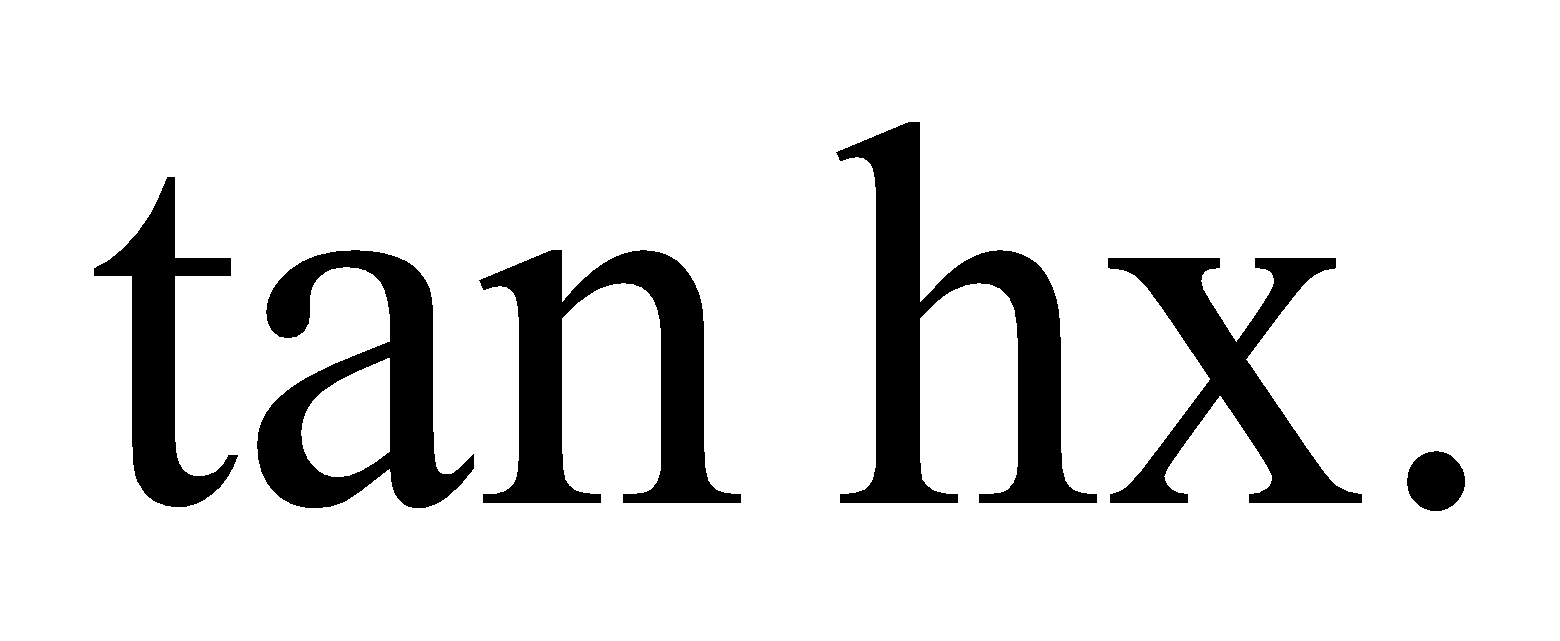1
(c) Write the expansion of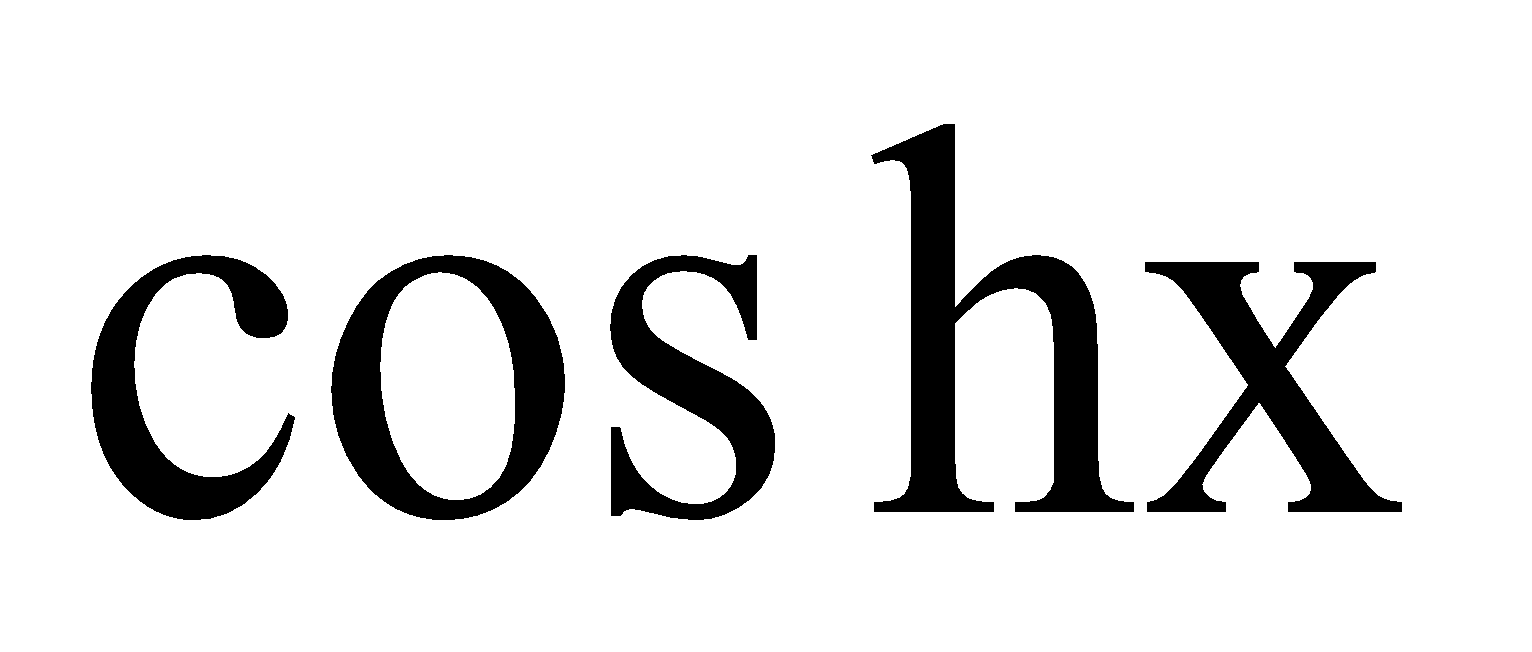in terms of x. 2
(d) Find the sum of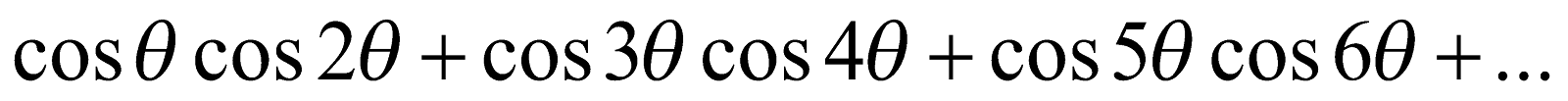up to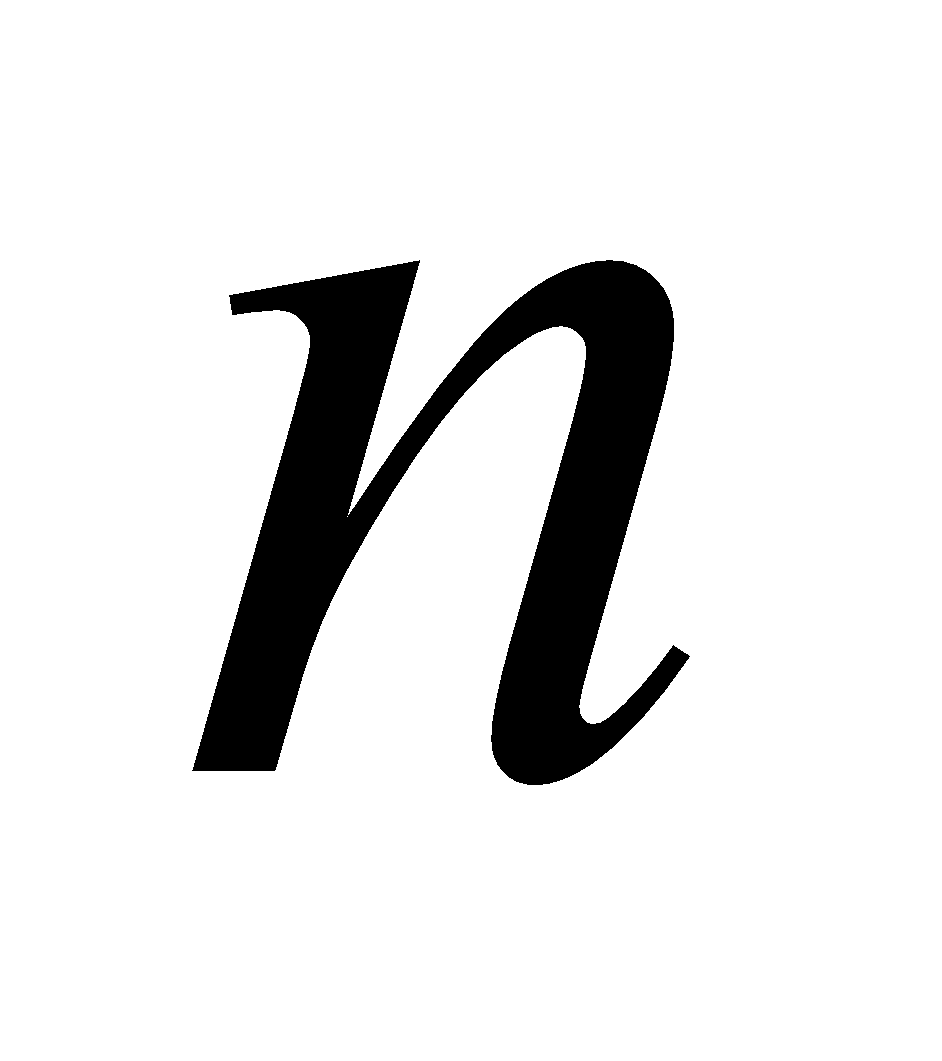terms. 4
Or
Separate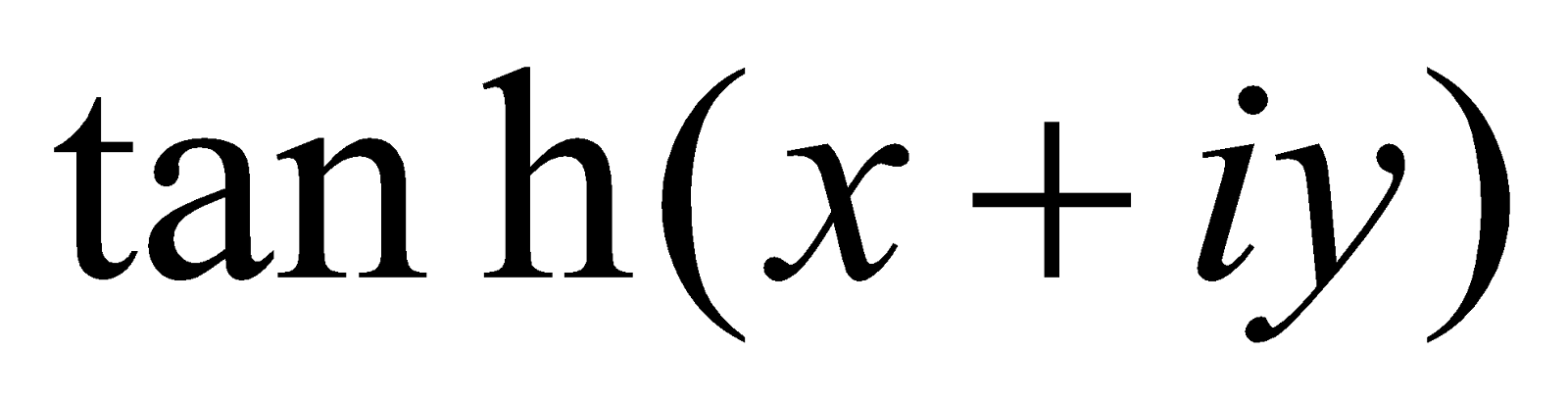into real and imaginary parts.

GROUP – C
(Vector Calculus)

8. (a) Choose the correct answer: 1
A vector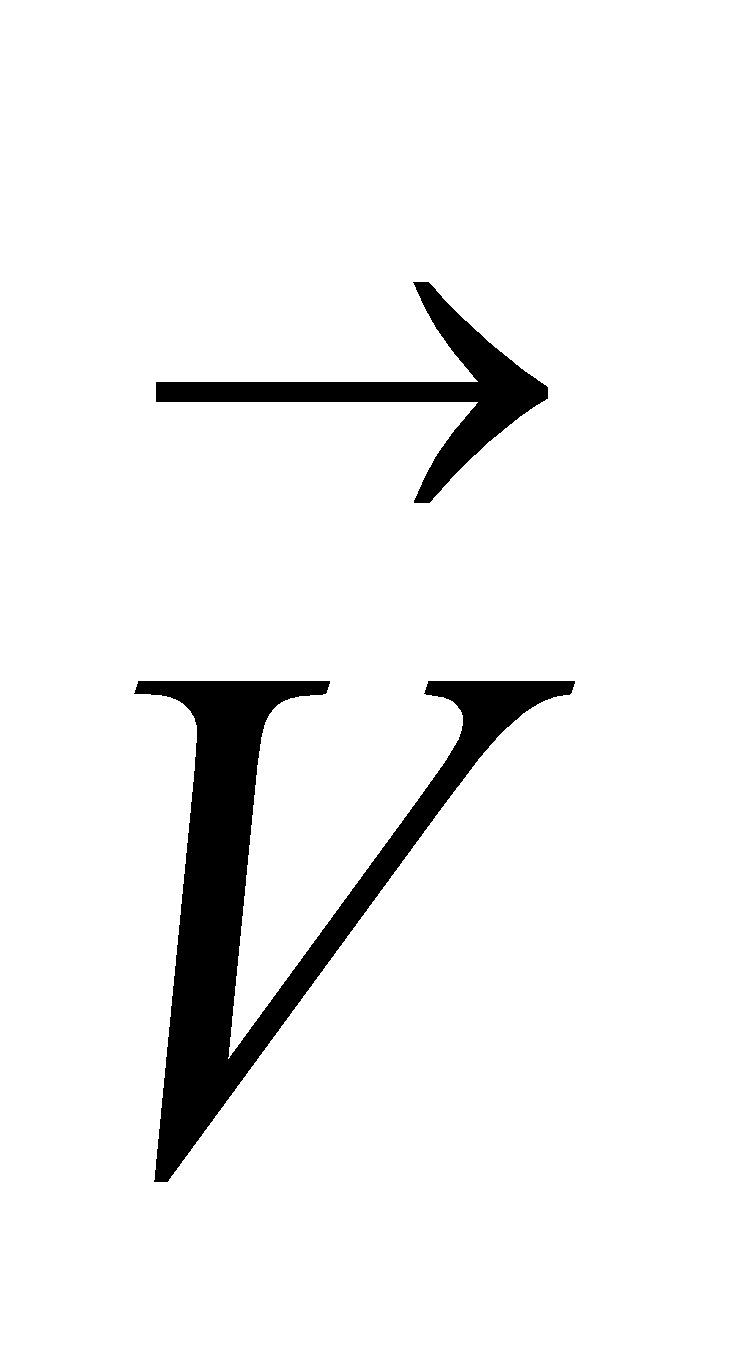is a solenoidal vector of
1.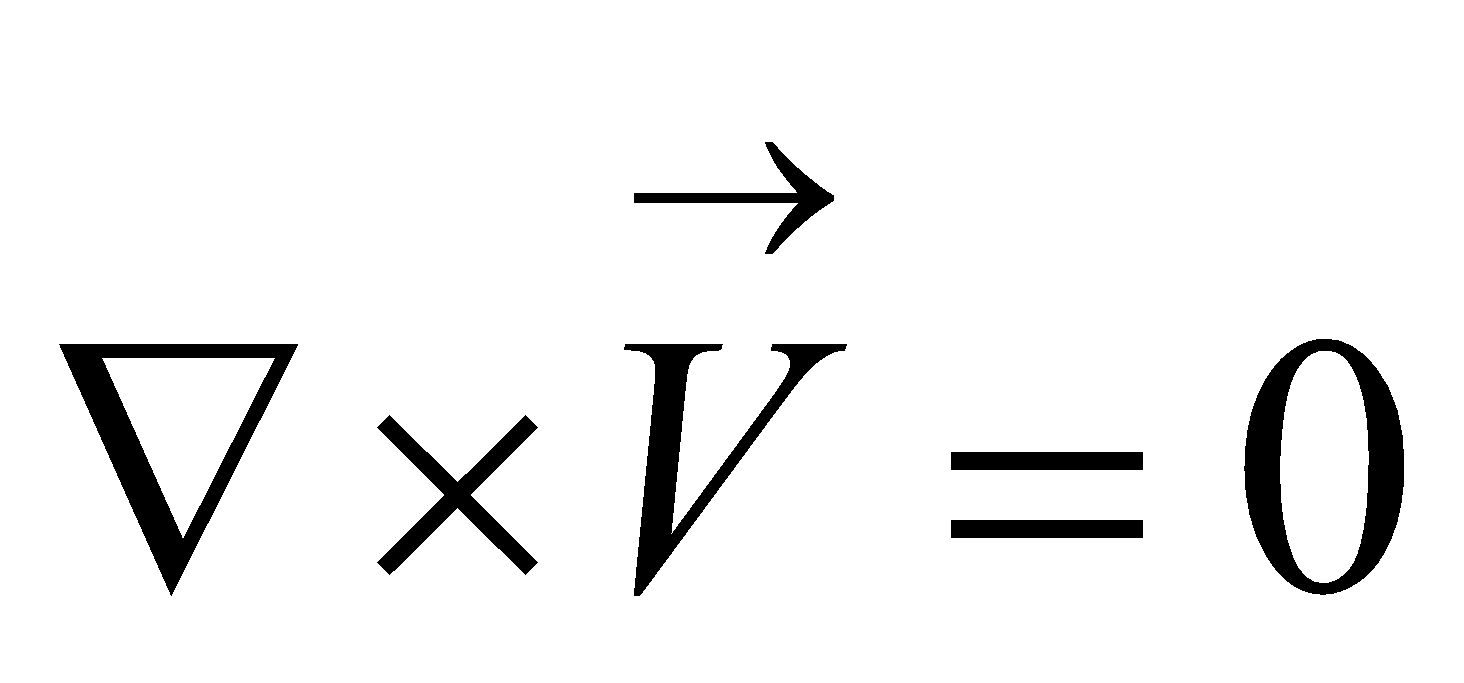2.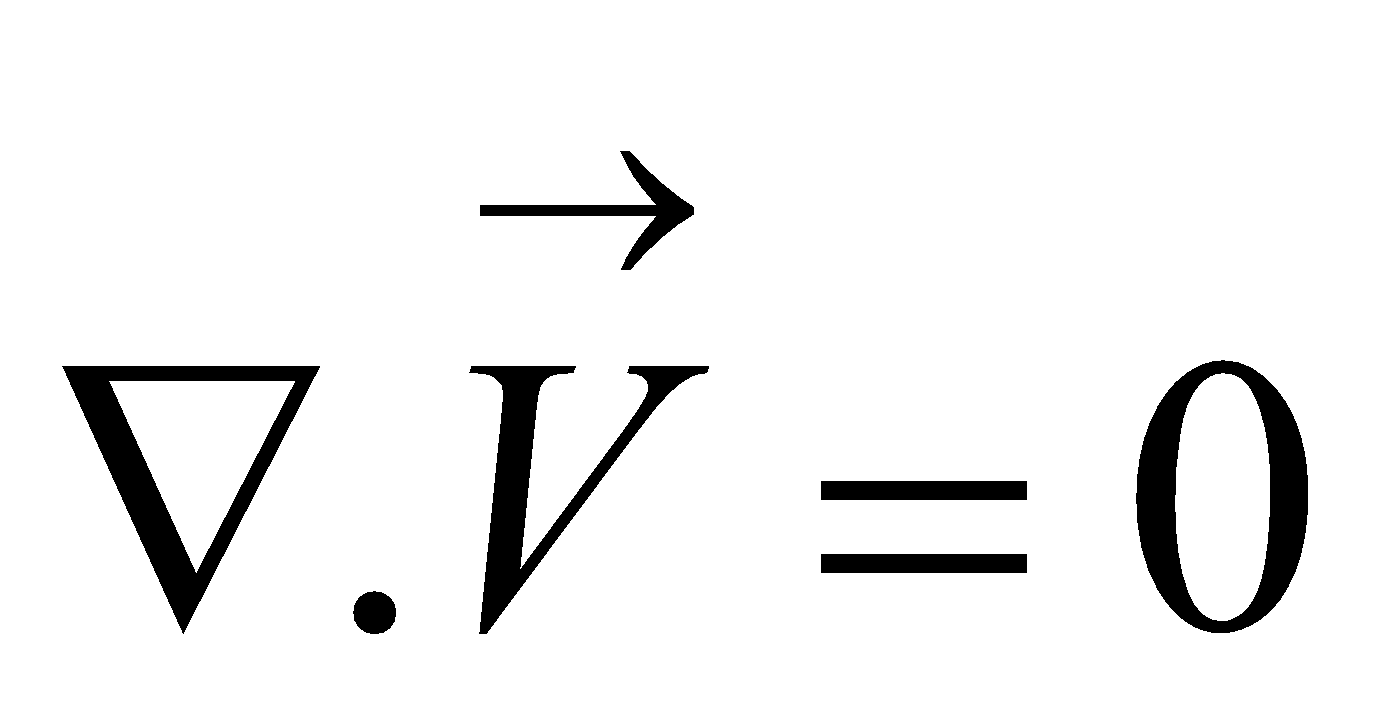3.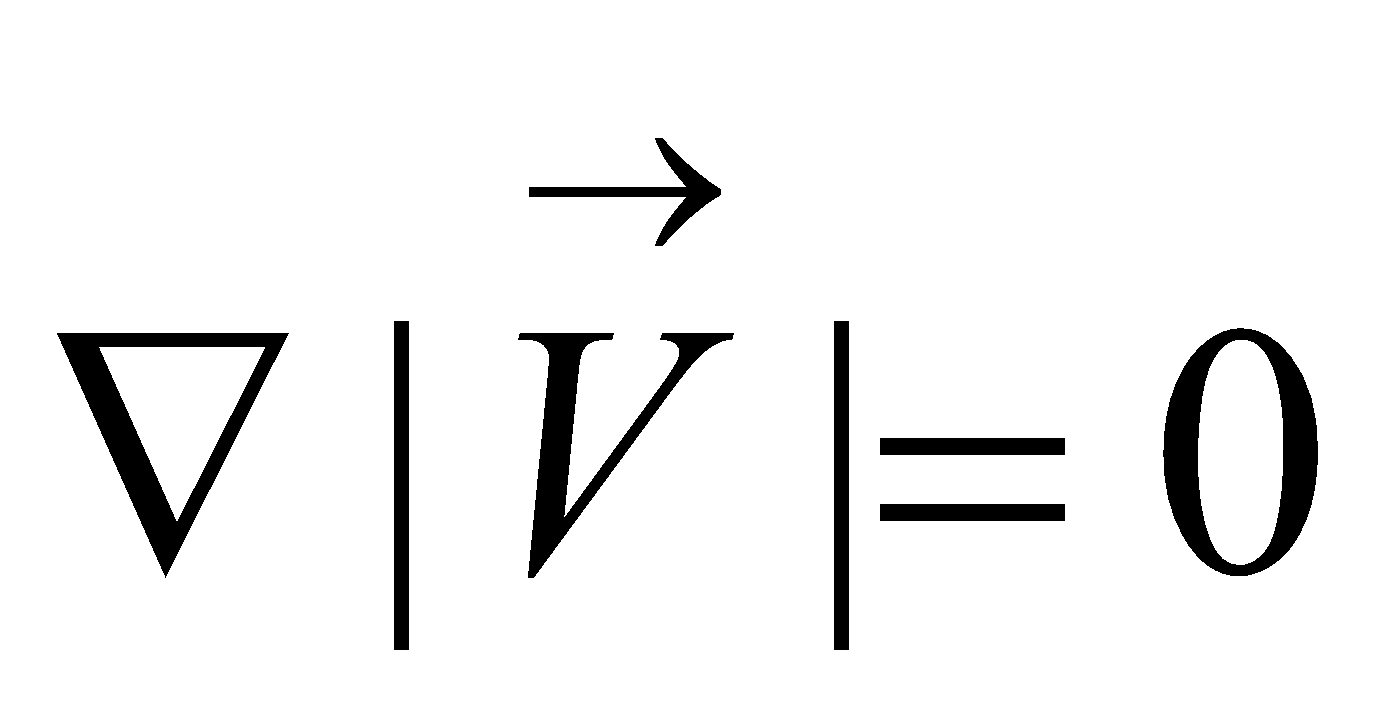4.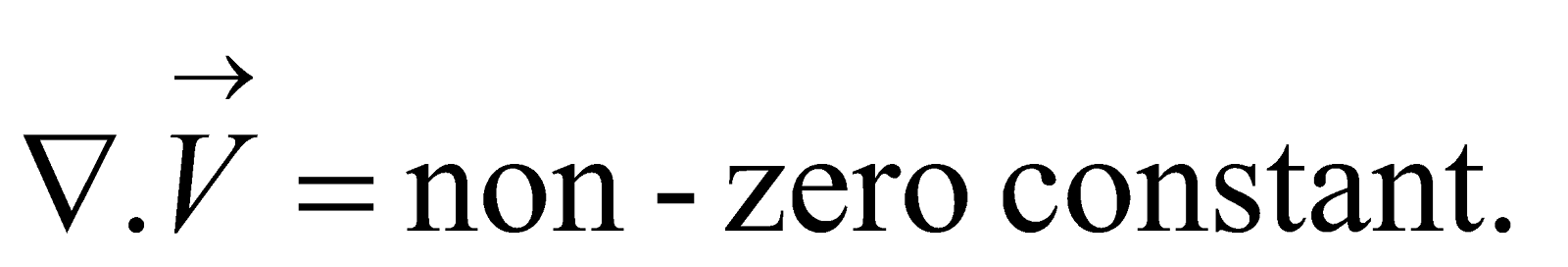(b) Find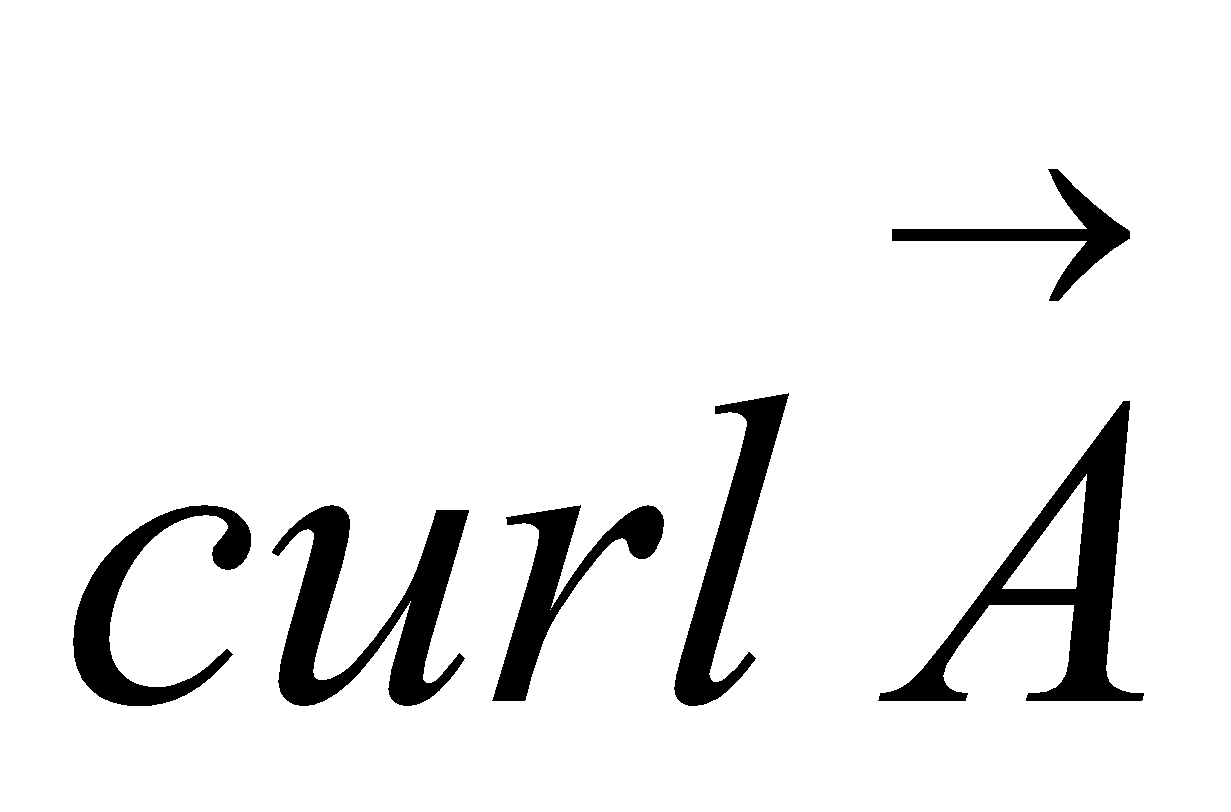, where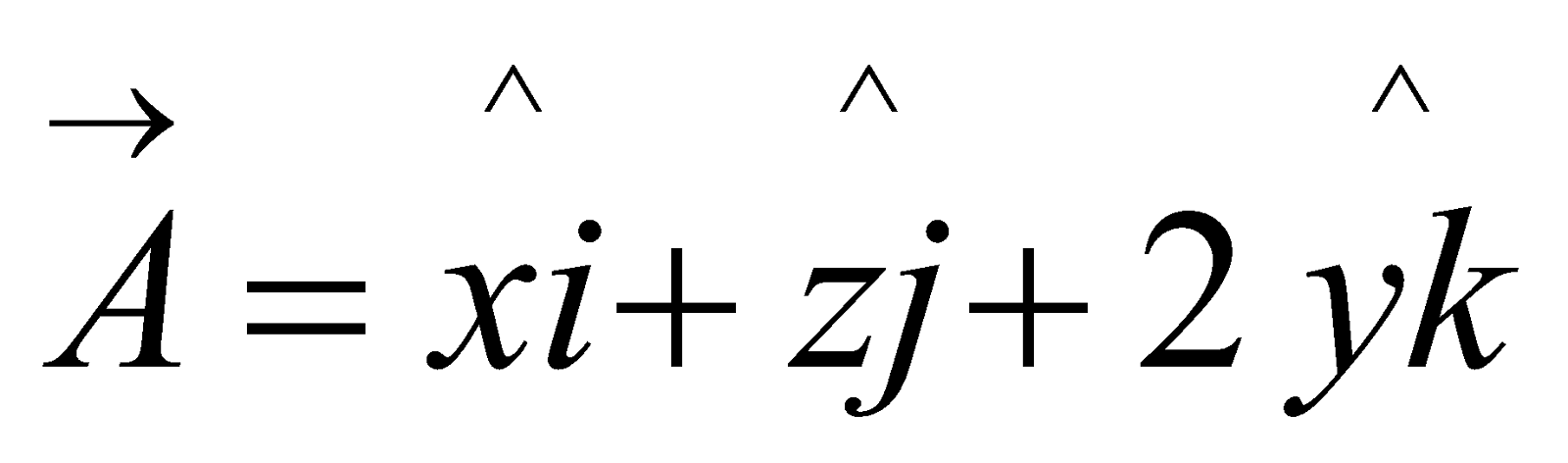2
(c) Show that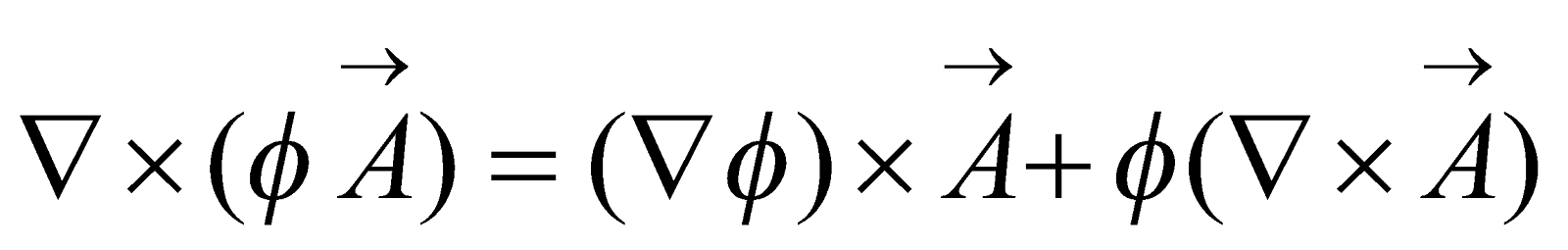, where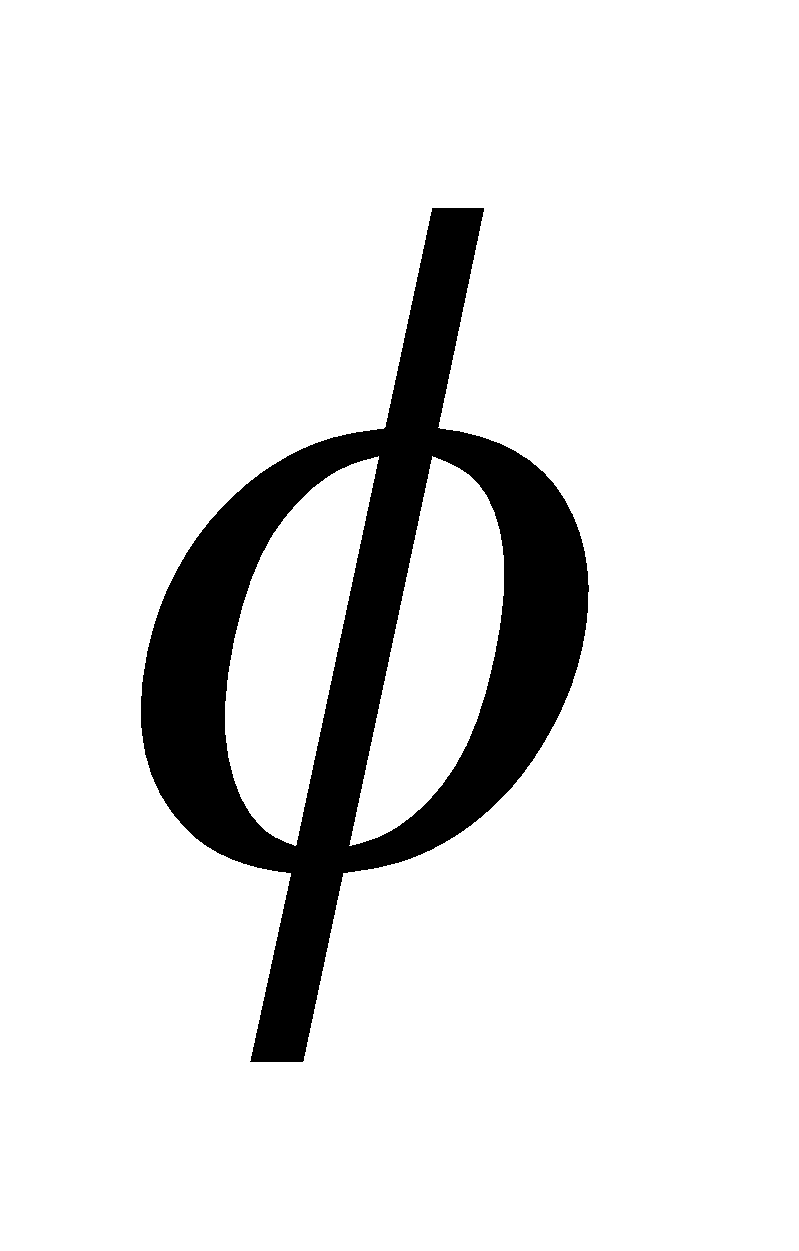is a scalar function and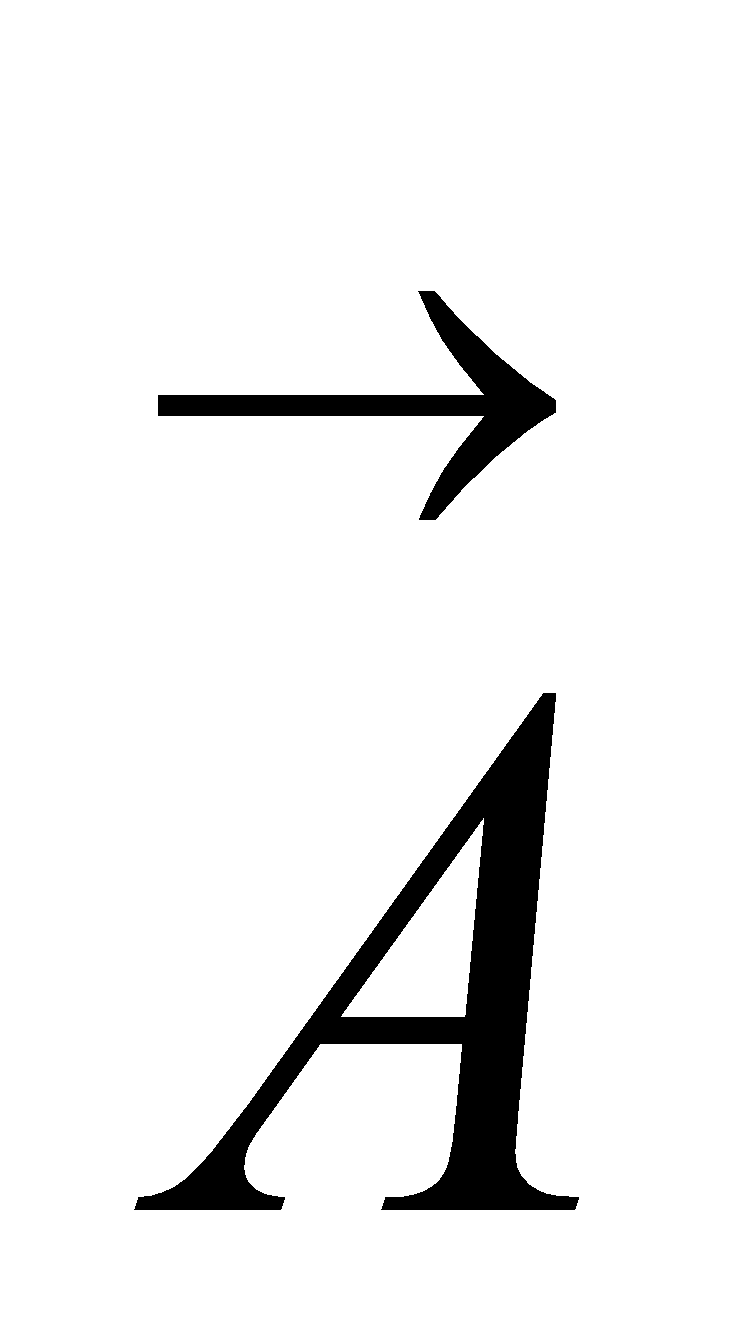is a vector function. 5
Or
Show that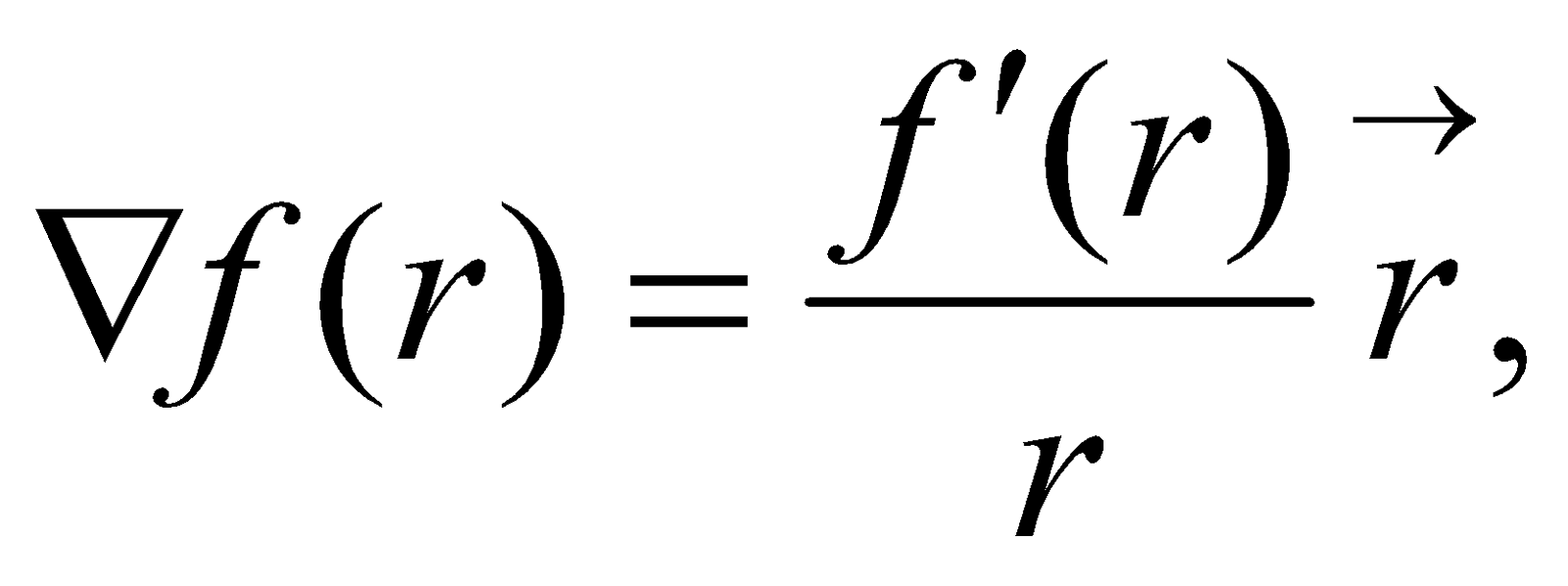where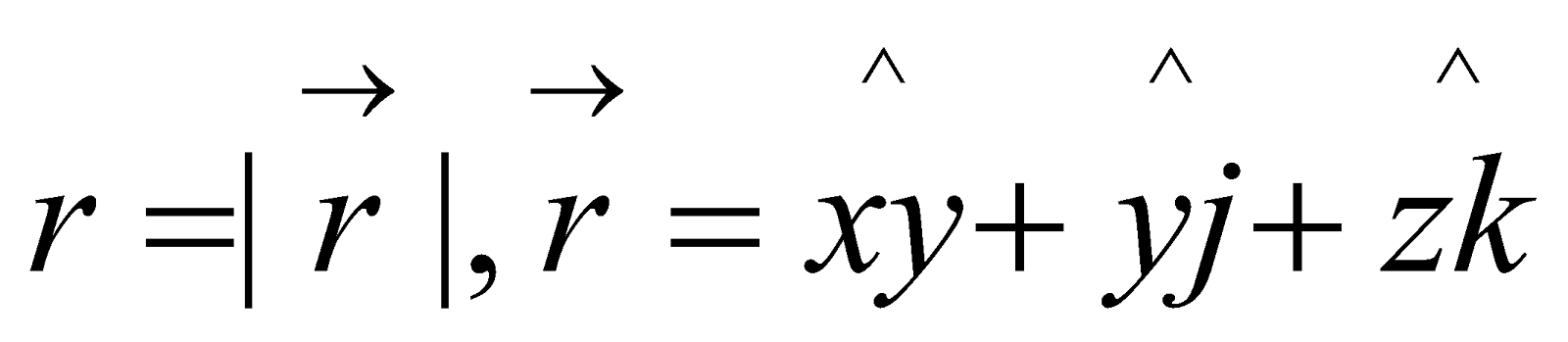(d) Show that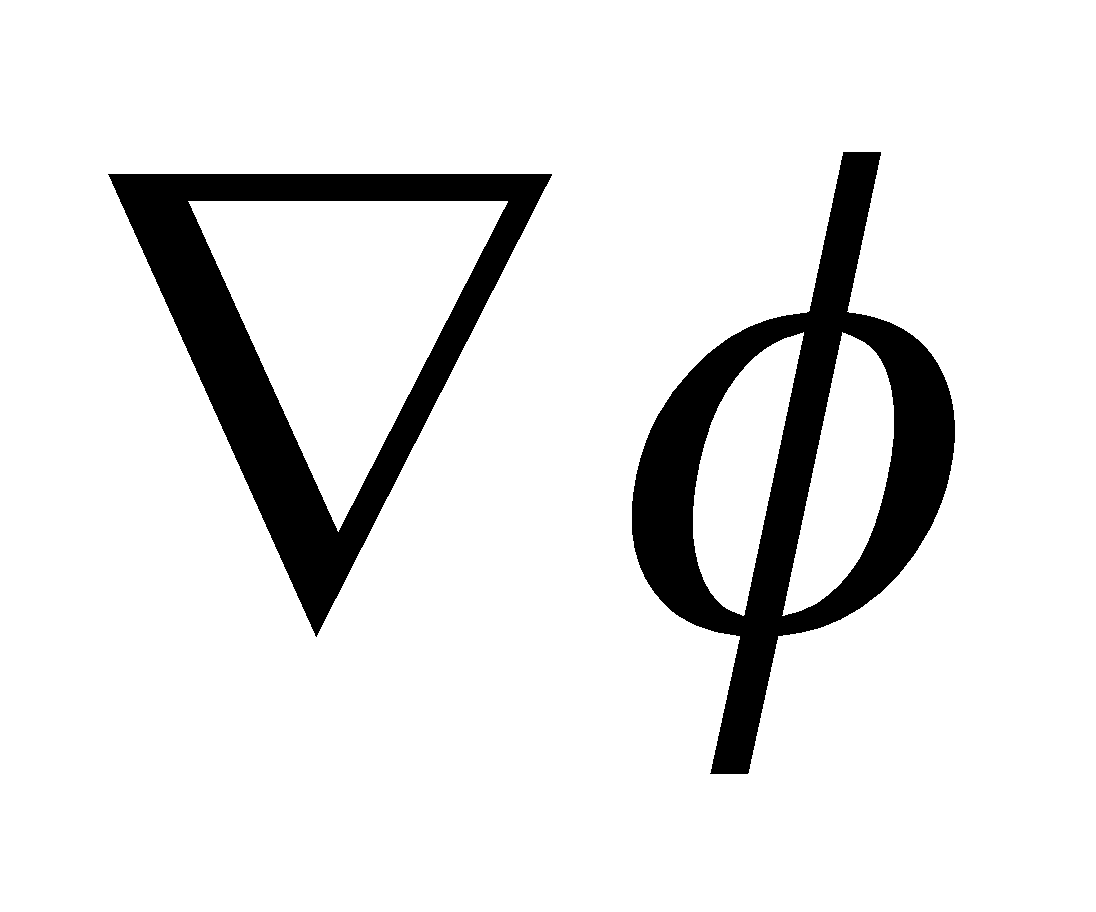is a vector perpendicular to the surface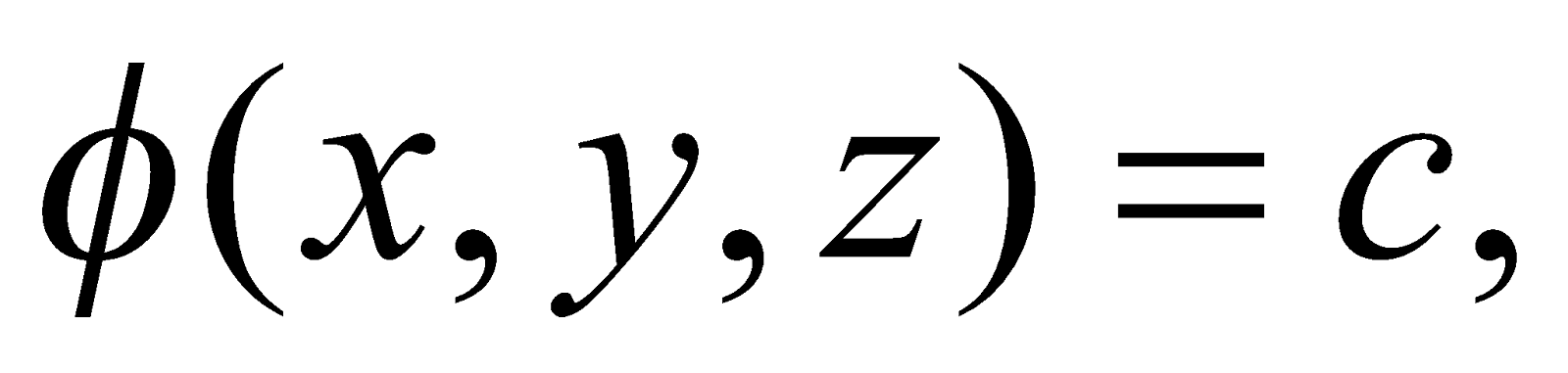where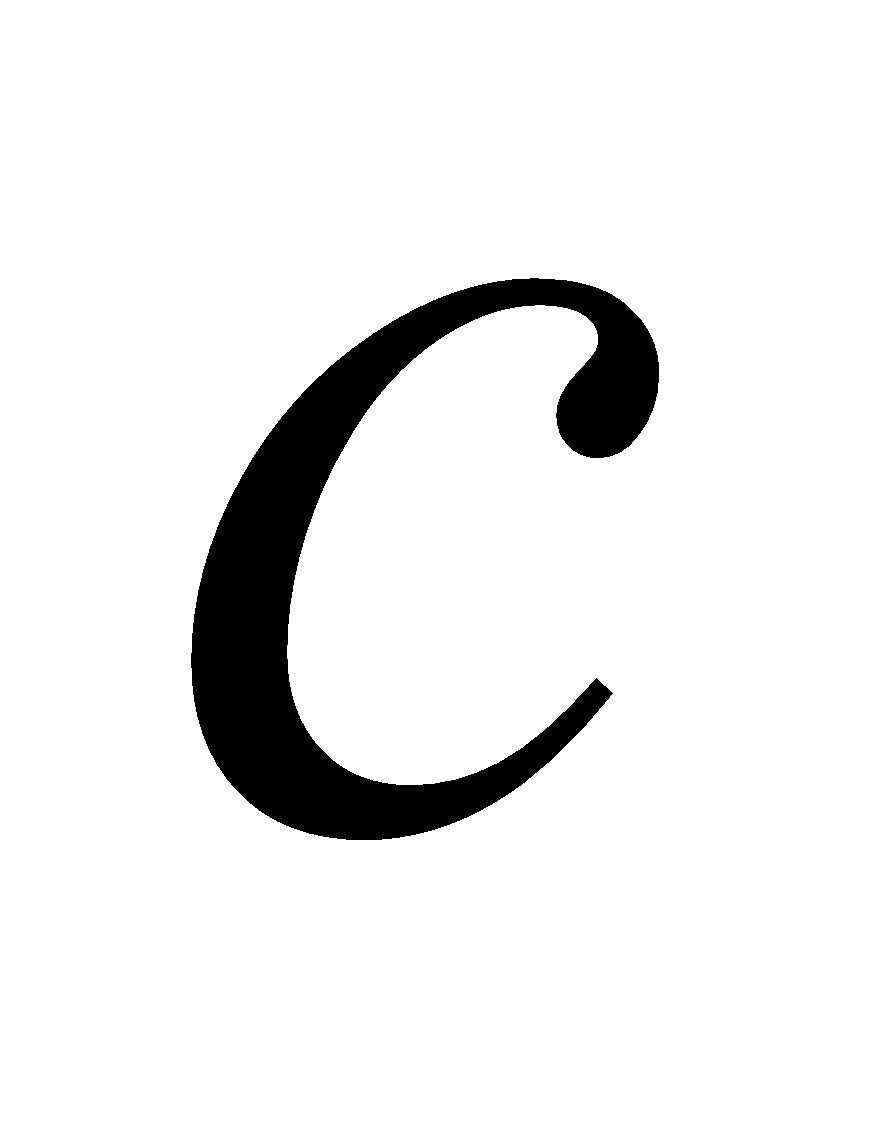is a constant. 3
(e) Evaluate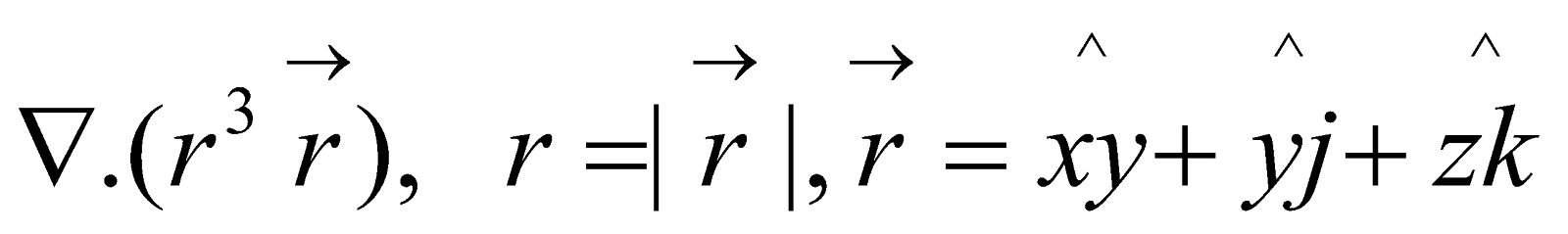4
Or
Find the directional derivative of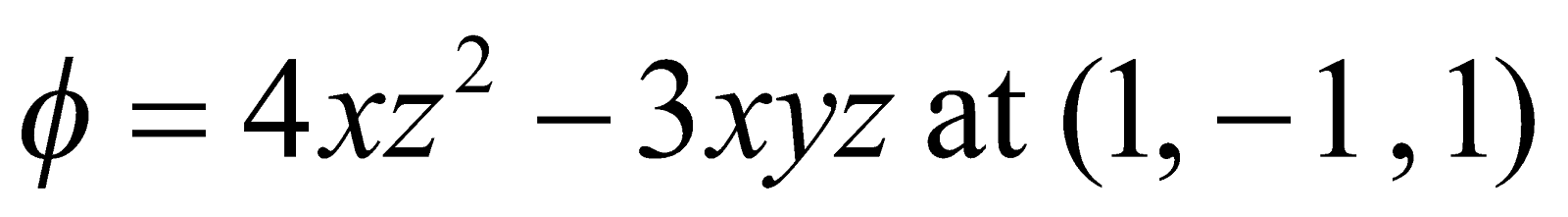in the direction of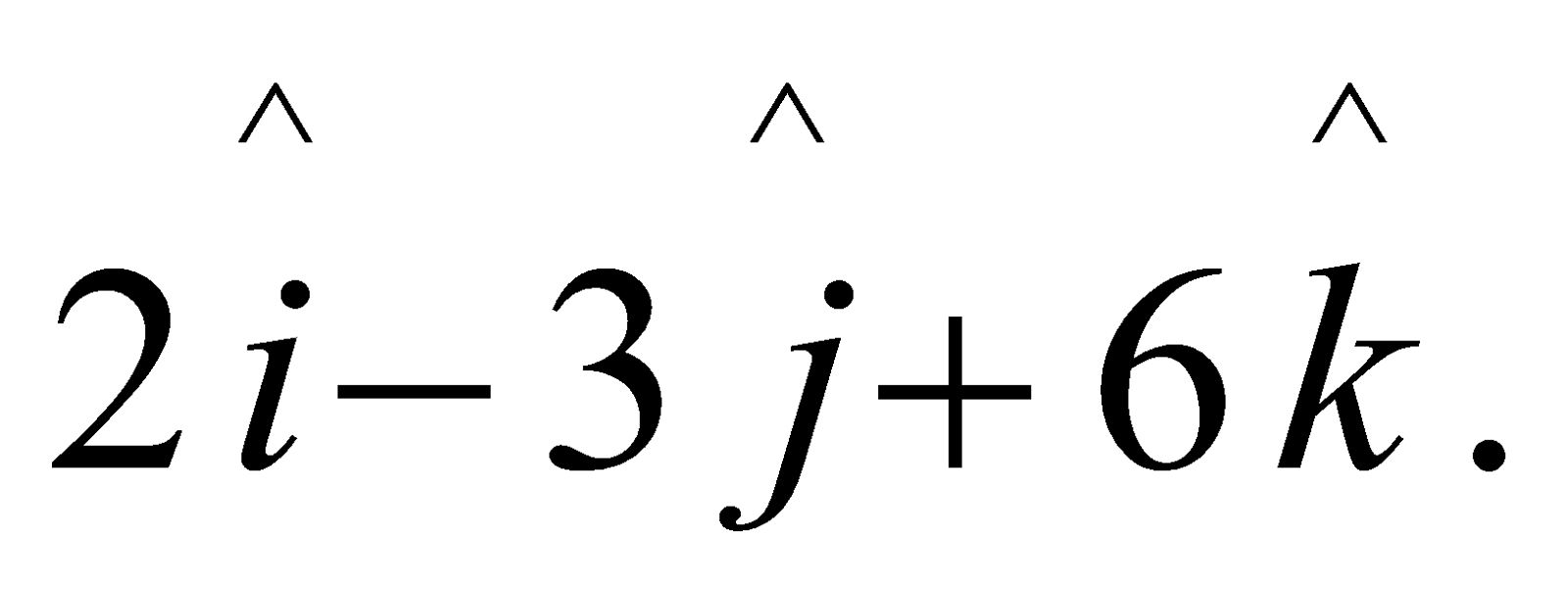***